# JP4150032B2 - Head position control method, head position control device, and disk device - Google Patents

## Info

Publication number
JP4150032B2
JP4150032B2 JP2005187029A JP2005187029A JP4150032B2 JP 4150032 B2 JP4150032 B2 JP 4150032B2 JP 2005187029 A JP2005187029 A JP 2005187029A JP 2005187029 A JP2005187029 A JP 2005187029A JP 4150032 B2 JP4150032 B2 JP 4150032B2
Authority
JP
Japan
Prior art keywords
external vibration
vibration suppression
value
control
estimated
Prior art date
Legal status (The legal status is an assumption and is not a legal conclusion. Google has not performed a legal analysis and makes no representation as to the accuracy of the status listed.)
Expired - Fee Related
Application number
JP2005187029A
Other languages
Japanese (ja)
Other versions
JP2007004946A (en
Inventor

Original Assignee

Priority date (The priority date is an assumption and is not a legal conclusion. Google has not performed a legal analysis and makes no representation as to the accuracy of the date listed.)
Filing date
Publication date
Application filed by 富士通株式会社 filed Critical 富士通株式会社
Priority to JP2005187029A priority Critical patent/JP4150032B2/en
Publication of JP2007004946A publication Critical patent/JP2007004946A/en
Application granted granted Critical
Publication of JP4150032B2 publication Critical patent/JP4150032B2/en
Expired - Fee Related legal-status Critical Current
Anticipated expiration legal-status Critical

• 230000001629 suppression Effects 0.000 claims description 230
• 230000003044 adaptive Effects 0.000 claims description 159
• 230000000875 corresponding Effects 0.000 claims description 27
• 230000000737 periodic Effects 0.000 claims description 27
• 230000003111 delayed Effects 0.000 claims description 26
• 230000001934 delay Effects 0.000 claims description 11
• 239000000284 extracts Substances 0.000 claims description 10
• 230000001276 controlling effects Effects 0.000 claims 3
• 238000004364 calculation methods Methods 0.000 description 73
• 238000000034 methods Methods 0.000 description 16
• 239000011159 matrix materials Substances 0.000 description 10
• 238000004088 simulation Methods 0.000 description 8
• 238000005094 computer simulation Methods 0.000 description 2
• 238000006243 chemical reaction Methods 0.000 description 1
• 239000000203 mixtures Substances 0.000 description 1
• 238000005070 sampling Methods 0.000 description 1

## Images

•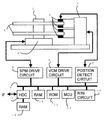•••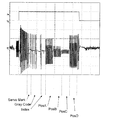•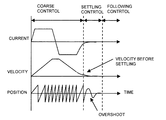•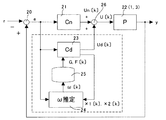•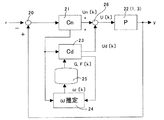•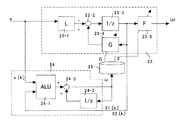•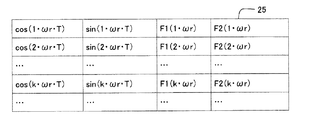••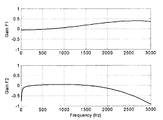•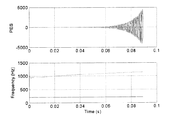•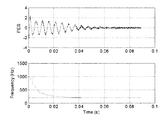•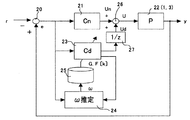•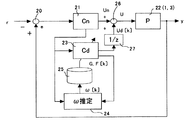•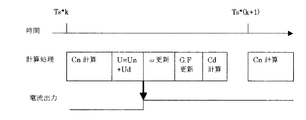•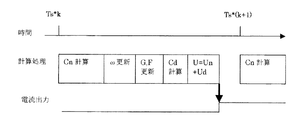••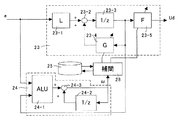•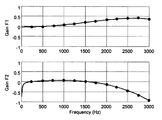•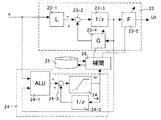••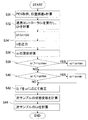•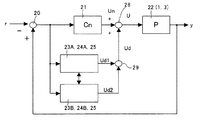•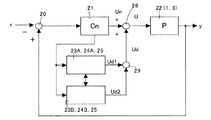•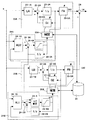•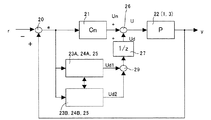•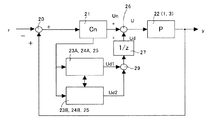•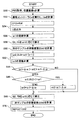•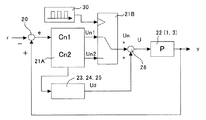•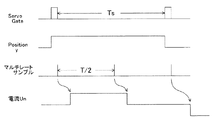•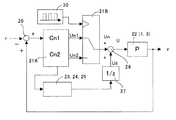•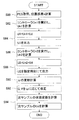•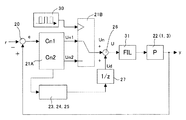•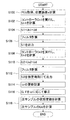••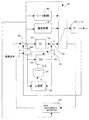•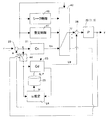••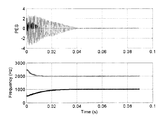•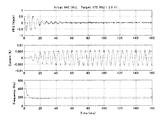•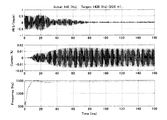•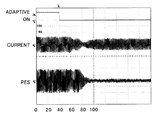••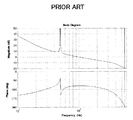## Classifications

• GPHYSICS
• G11INFORMATION STORAGE
• G11BINFORMATION STORAGE BASED ON RELATIVE MOVEMENT BETWEEN RECORD CARRIER AND TRANSDUCER
• G11B5/00Recording by magnetisation or demagnetisation of a record carrier; Reproducing by magnetic means; Record carriers therefor
• G11B5/48Disposition or mounting of heads or head supports relative to record carriers ; arrangements of heads, e.g. for scanning the record carrier to increase the relative speed
• G11B5/54Disposition or mounting of heads or head supports relative to record carriers ; arrangements of heads, e.g. for scanning the record carrier to increase the relative speed with provision for moving the head into or out of its operative position or across tracks
• G11B5/55Track change, selection or acquisition by displacement of the head
• G11B5/5521Track change, selection or acquisition by displacement of the head across disk tracks
• G11B5/5582Track change, selection or acquisition by displacement of the head across disk tracks system adaptation for working during or after external perturbation, e.g. in the presence of a mechanical oscillation caused by a shock
• GPHYSICS
• G11INFORMATION STORAGE
• G11BINFORMATION STORAGE BASED ON RELATIVE MOVEMENT BETWEEN RECORD CARRIER AND TRANSDUCER
• G11B21/00Head arrangements not specific to the method of recording or reproducing
• G11B21/02Driving or moving of heads
• G11B21/08Track changing or selecting during transducing operation
• G11B21/083Access to indexed tracks or parts of continuous track on discs
• G11B21/085Access to indexed tracks or parts of continuous track on discs with track following of accessed part
• GPHYSICS
• G11INFORMATION STORAGE
• G11BINFORMATION STORAGE BASED ON RELATIVE MOVEMENT BETWEEN RECORD CARRIER AND TRANSDUCER
• G11B5/00Recording by magnetisation or demagnetisation of a record carrier; Reproducing by magnetic means; Record carriers therefor
• G11B5/48Disposition or mounting of heads or head supports relative to record carriers ; arrangements of heads, e.g. for scanning the record carrier to increase the relative speed
• G11B5/58Disposition or mounting of heads or head supports relative to record carriers ; arrangements of heads, e.g. for scanning the record carrier to increase the relative speed with provision for moving the head for the purpose of maintaining alignment of the head relative to the record carrier during transducing operation, e.g. to compensate for surface irregularities of the latter or for track following
• G11B5/596Disposition or mounting of heads or head supports relative to record carriers ; arrangements of heads, e.g. for scanning the record carrier to increase the relative speed with provision for moving the head for the purpose of maintaining alignment of the head relative to the record carrier during transducing operation, e.g. to compensate for surface irregularities of the latter or for track following for track following on disks
• G11B5/59688Servo signal format patterns or signal processing thereof, e.g. dual, tri, quad, burst signal patterns
• GPHYSICS
• G11INFORMATION STORAGE
• G11BINFORMATION STORAGE BASED ON RELATIVE MOVEMENT BETWEEN RECORD CARRIER AND TRANSDUCER
• G11B7/00Recording or reproducing by optical means, e.g. recording using a thermal beam of optical radiation by modifying optical properties or the physical structure, reproducing using an optical beam at lower power by sensing optical properties; Record carriers therefor
• G11B7/08Disposition or mounting of heads or light sources relatively to record carriers
• G11B7/085Disposition or mounting of heads or light sources relatively to record carriers with provision for moving the light beam into, or out of, its operative position or across tracks, otherwise than during the transducing operation, e.g. for adjustment or preliminary positioning or track change or selection
• G11B7/08505Methods for track change, selection or preliminary positioning by moving the head
• GPHYSICS
• G11INFORMATION STORAGE
• G11BINFORMATION STORAGE BASED ON RELATIVE MOVEMENT BETWEEN RECORD CARRIER AND TRANSDUCER
• G11B11/00Recording on or reproducing from the same record carrier wherein for these two operations the methods are covered by different main groups of groups G11B3/00 - G11B7/00 or by different subgroups of group G11B9/00; Record carriers therefor
• G11B11/10Recording on or reproducing from the same record carrier wherein for these two operations the methods are covered by different main groups of groups G11B3/00 - G11B7/00 or by different subgroups of group G11B9/00; Record carriers therefor using recording by magnetic means or other means for magnetisation or demagnetisation of a record carrier, e.g. light induced spin magnetisation; Demagnetisation by thermal or stress means in the presence or not of an orienting magnetic field
• G11B11/105Recording on or reproducing from the same record carrier wherein for these two operations the methods are covered by different main groups of groups G11B3/00 - G11B7/00 or by different subgroups of group G11B9/00; Record carriers therefor using recording by magnetic means or other means for magnetisation or demagnetisation of a record carrier, e.g. light induced spin magnetisation; Demagnetisation by thermal or stress means in the presence or not of an orienting magnetic field using a beam of light or a magnetic field for recording by change of magnetisation and a beam of light for reproducing, i.e. magneto-optical, e.g. light-induced thermo-magnetic recording, spin magnetisation recording, Kerr or Faraday effect reproducing
• G11B11/1055Disposition or mounting of transducers relative to record carriers
• G11B11/10556Disposition or mounting of transducers relative to record carriers with provision for moving or switching or masking the transducers in or out of their operative position
• G11B11/10563Access of indexed parts
• GPHYSICS
• G11INFORMATION STORAGE
• G11BINFORMATION STORAGE BASED ON RELATIVE MOVEMENT BETWEEN RECORD CARRIER AND TRANSDUCER
• G11B5/00Recording by magnetisation or demagnetisation of a record carrier; Reproducing by magnetic means; Record carriers therefor
• G11B5/48Disposition or mounting of heads or head supports relative to record carriers ; arrangements of heads, e.g. for scanning the record carrier to increase the relative speed
• G11B5/58Disposition or mounting of heads or head supports relative to record carriers ; arrangements of heads, e.g. for scanning the record carrier to increase the relative speed with provision for moving the head for the purpose of maintaining alignment of the head relative to the record carrier during transducing operation, e.g. to compensate for surface irregularities of the latter or for track following
• G11B5/596Disposition or mounting of heads or head supports relative to record carriers ; arrangements of heads, e.g. for scanning the record carrier to increase the relative speed with provision for moving the head for the purpose of maintaining alignment of the head relative to the record carrier during transducing operation, e.g. to compensate for surface irregularities of the latter or for track following for track following on disks
• G11B5/59627Aligning for runout, eccentricity or offset compensation
• GPHYSICS
• G11INFORMATION STORAGE
• G11BINFORMATION STORAGE BASED ON RELATIVE MOVEMENT BETWEEN RECORD CARRIER AND TRANSDUCER
• G11B7/00Recording or reproducing by optical means, e.g. recording using a thermal beam of optical radiation by modifying optical properties or the physical structure, reproducing using an optical beam at lower power by sensing optical properties; Record carriers therefor
• G11B7/08Disposition or mounting of heads or light sources relatively to record carriers
• G11B7/09Disposition or mounting of heads or light sources relatively to record carriers with provision for moving the light beam or focus plane for the purpose of maintaining alignment of the light beam relative to the record carrier during transducing operation, e.g. to compensate for surface irregularities of the latter or for track following
• G11B7/0941Methods and circuits for servo gain or phase compensation during operation
• GPHYSICS
• G11INFORMATION STORAGE
• G11BINFORMATION STORAGE BASED ON RELATIVE MOVEMENT BETWEEN RECORD CARRIER AND TRANSDUCER
• G11B7/00Recording or reproducing by optical means, e.g. recording using a thermal beam of optical radiation by modifying optical properties or the physical structure, reproducing using an optical beam at lower power by sensing optical properties; Record carriers therefor
• G11B7/08Disposition or mounting of heads or light sources relatively to record carriers
• G11B7/09Disposition or mounting of heads or light sources relatively to record carriers with provision for moving the light beam or focus plane for the purpose of maintaining alignment of the light beam relative to the record carrier during transducing operation, e.g. to compensate for surface irregularities of the latter or for track following
• G11B7/0946Disposition or mounting of heads or light sources relatively to record carriers with provision for moving the light beam or focus plane for the purpose of maintaining alignment of the light beam relative to the record carrier during transducing operation, e.g. to compensate for surface irregularities of the latter or for track following specially adapted for operation during external perturbations not related to the carrier or servo beam, e.g. vibration

## Description

The present invention relates to a head position control method and a disk device for a disk apparatus for suppressing head position shift due to disturbance, and more particularly to a head position control method and a disk apparatus for suppressing head position shift due to external vibration.

In a disk apparatus that records and reproduces data with a head on a rotating storage medium (for example, a disk medium), the head is positioned on a desired track of the storage medium, and data is read / written on the track. In such a disk device, for example, a magnetic disk device or an optical disk device, accurately positioning the head on the target track is extremely important for improving the recording density.

As one of the factors that hinder this positioning, there is an eccentricity caused by the fact that the center of the concentric servo signal on the disk is different from the rotation center of the motor. As a technique for correcting this eccentricity, there is a control method using an observer (see, for example, Patent Document 1).

The eccentricity generates a sine wave-like position fluctuation synchronized with an integral multiple of the rotation frequency. The observer control method can suppress such sinusoidal position fluctuation and accurately position the target track. However, in such eccentricity correction, the frequency to be corrected must be known in advance. For example, it must be an integral multiple of the number of revolutions, 1 or 2 times.

A second factor that hinders positioning is vibration applied from the outside of the disk device. This vibration has various waveforms. Here, a method for dealing with a sinusoidal vibration will be examined. By applying the eccentricity correction control, it is possible to deal with frequencies other than an integral multiple of the rotational speed.

The above-described conventional configuration is based on the premise that the disturbance frequency is known. However, what kind of vibration is applied to external vibration is unknown at the time of design of the control system, and its frequency cannot be grasped in advance. Therefore, some unknown frequency detection means is required, and if even the frequency can be detected, it is possible to suppress the position fluctuation due to the external vibration by using the control method shown in Patent Document 1 described above.

FIG. 44 is a configuration diagram of a control system for detecting a conventional disturbance frequency and suppressing a sinusoidal disturbance having a predetermined frequency. A position error e between the target position r and the observation position y is calculated by the calculator 100 and input to the controller 102 (Cn) that performs feedback control. The controller 102 outputs a control current value Un by known PID control, PI control + LeadLag, and observer control.

A frequency estimator (ω estimation) 106 for estimating the frequency of disturbance and a compensator (Cd) 104 for suppressing disturbance at a specific frequency are added to the controller 102 by adaptive control. U that is the sum of the output Un of the controller 102 (Cn) and the output Ud of the compensator 104 (Cd) is supplied to the control object 103 (P). This frequency estimator 106 estimates the angular frequency ω (= 2πf) of the disturbance based on the position error e, and introduces it into the transfer function of disturbance frequency suppression of the compensator 104. The compensator 104 calculates a recurrence formula (adaptive control formula) of a sine wave from the position error e and the estimated angular frequency ω, and calculates a compensation current output Ud.

In this way, the conventional eccentricity correction control detects the disturbance frequency and suppresses the unknown frequency so as to cope with the disturbance of the unknown frequency within a certain range (see, for example, Non-Patent Document 2). As a method for estimating the unknown frequency and suppressing disturbance of the unknown frequency, an assumption of a recurrence formula of a sine wave (see, for example, Non-Patent Document 1), an adaptive law based on the error signal described above is used. There has been proposed one that introduces and corrects a drive amount to be controlled (see, for example, Non-Patent Document 2). Further, it has also been proposed that an unknown frequency is estimated from an error signal, a disturbance suppression signal at a position level is generated, the error signal is corrected, and input to a controller (for example, see Patent Document 2).

For example, as shown in the example of the open loop characteristics of the system of FIG. 45, the zero cross frequency of the open loop characteristics is set to 1000 Hz, and the target disturbance frequency is set to 500 Hz. This is a characteristic represented by the expression (Cn + Cd) P in FIG. In this way, disturbance can be suppressed by setting the gain of the open loop characteristic at a specific frequency (here, 500 Hz) high.
Japanese Patent No. 3,460,795 US Pat. No. 6,762,902 IEEJ Technical Meeting Industrial Measurement Society IIC-04-70 "Frequency Tracking Peak Filter" (issued September 10, 2004) Proceedings of the 40th IEEE Conference on Decision and Control, pp. 4909-4914 (issued in December 2001)

In recent years, such a disk device, in particular, a hard disk drive (HDD), is mounted on a mobile device such as a portable personal computer, a portable terminal, a cellular phone, and a portable AV device. When used in such an environment, the frequency of the unknown disturbance is unspecified and needs to be adapted to a wide range of disturbance frequencies.

For this reason, when adding a conventional compensator for suppressing external vibration to an existing controller, it is necessary to appropriately set a coefficient corresponding to the frequency of external vibration in the suppression signal generation equation. For example, in the system of FIG. 44, it is necessary to adjust the gain and phase of the transfer function (adaptive law) of the compensator 104 that suppresses external vibration. If this adjustment is not appropriate, the control system becomes unstable.

In the conventional various disturbance suppression control methods described above, coefficients such as gain and phase are determined in advance empirically or predictively based on the design theory of the feedback system, and the generation formula is fixed. With such a coefficient setting, disturbance suppression by a certain range of disturbance frequencies (for example, a predicted frequency range of the servo system) is possible.

However, when the disk device is used in the mobile environment as described above, the range of the unknown disturbance frequency varies depending on the use situation and is difficult to predict. In the conventional technique, since the frequency range in which the adaptive control of the disturbance can be followed is limited, the adjusted gain and phase are not appropriate in the use environment described above, and the control system may become unstable. In addition, the magnitude of the disturbance cannot be specified, the control system becomes unstable, the error is amplified, and there is a possibility of divergence.

Therefore, an object of the present invention is to provide a head position control method, a head position control device, and a disk device for realizing disturbance suppression control adapted to a wider range of disturbance frequencies.

Another object of the present invention is to provide a head position control method, a head position control device, and a disk device for realizing disturbance suppression control even when used in a mobile environment.

Another object of the present invention is to provide a head position control method, a head position control device, and a disk device for realizing disturbance suppression control adapted to a wider range of disturbance frequencies and disturbance amplitudes.

Another object of the present invention is to provide a head position control method, a head position control device, and a disk device for realizing disturbance suppression control with high accuracy and adapted to a wider range of disturbance frequencies.

In order to achieve this object, the present invention is a head positioning control method in which the head is positioned on a desired track of the disk by an actuator in accordance with a position signal read by the head from the disk. The control method includes a step of obtaining a control value of the actuator in accordance with a position error between a target position and a current position obtained from the position signal, and a stepwise external signal according to an adaptive law from a signal based on the position error. Estimating a vibration frequency; estimating a rotation vector of a periodic disturbance from a signal based on the position error; obtaining an external vibration suppression control value using an external vibration suppression constant; and the successive estimation depending on the external vibration frequencies, the steps of sequentially correcting the constant for external vibration suppression, by adding the control value and the external vibration suppression control value, possess and generating a drive value of the actuator The external vibration suppression control value is further delayed by one sample, and the generating step is delayed by one sample with the control value in the current sample. By adding the external vibration suppression control value, comprising the step of generating a drive value of the actuator.

In the present invention, it is preferable that the estimation step includes a step of sequentially estimating an angular frequency of disturbance according to an adaptive law from a signal based on the position error, and the step of obtaining the external vibration suppression value includes: The rotation vector of the estimated periodic disturbance is converted into a current value as an external vibration suppression control value using an output gain as an external vibration suppression constant, and the correction step includes the sequentially estimated angle. A step of sequentially correcting the output gain in accordance with the frequency.

Further, in the present invention, it is preferable that the correction step refers to a table storing output gains for each of a plurality of angular frequency values by referring to the estimated angular frequency, takes out the corresponding output gain, and extracts the external gain. The method includes a step of updating an output gain as a vibration suppression constant.

Furthermore, in the present invention, it is preferable that the correction step includes referring to a table storing output gains for each of a plurality of angular frequency values by referring to the estimated angular frequency and extracting the two corresponding output gains. And calculating the output gain of the estimated angular frequency by interpolating the two output gains, and updating the output gain as the external vibration suppression constant.

Furthermore, in the present invention, preferably, the method further includes a step of delaying the external vibration suppression control value by one sample, and the generation step includes the control value in the current sample and the external vibration suppression control delayed by one sample. It includes a step of adding values to generate a drive value of the actuator.

In the present invention, it is preferable that the estimation step further includes a step of limiting the estimated angular frequency with an upper limit value and a lower limit value.

Furthermore, in the present invention, preferably, the estimation step, the correction step, the step of obtaining the external vibration suppression value, and the generation step are executed for each sample, and the step of obtaining the control value is performed by the one sample Run multiple times in between.

In the present invention, it is preferable that the estimation step, the correction step, and the step of obtaining the external vibration suppression value are performed for each of a plurality of external vibration frequencies, and the plurality of external vibration frequencies corresponding to the external vibration frequencies. And a step of adding a vibration suppression value.

In the present invention, it is preferable that the executing step further includes a step of correcting the one estimated external vibration frequency so that the estimated external vibration frequencies do not overlap.

In the present invention, since the external vibration frequency is estimated from the signal based on the position error by an adaptive law, an accurate external vibration frequency that has been integrated and compensated can be estimated, and the compensator is sequentially used by using the external vibration frequency. Therefore, it is possible to perform highly accurate follow-up control over a wide range of external vibration frequencies. In this way, according to the estimated value of the external vibration frequency, the operation of the compensator can always be kept optimal, and by correcting the compensator constant, tracking control can be performed for a wide range of external vibration frequencies. It becomes possible.

Hereinafter, the present invention will be described with reference to a disk device, a first embodiment of a positioning control system, a second embodiment of a positioning control system, a third embodiment of a positioning control system, and a fourth embodiment of a positioning control system. The fifth embodiment of the positioning control system, the sixth embodiment of the positioning control system, the seventh embodiment of the positioning control system, the example, and other embodiments will be described in this order. In the present invention, a magnetic disk device (hard disk drive) will be described below as an example. However, the technology described in the present invention can also be applied to other disk devices, for example, optical disk devices such as CD-ROM and DVD-ROM, and magneto-optical disk devices such as MO and MD.

[Disk unit]
1 is a configuration diagram of a disk storage device according to an embodiment of the present invention, FIG. 2 is a layout diagram of position signals of the magnetic disk of FIG. 1, and FIG. 3 is a position signal of the magnetic disk of FIGS. FIG. 4 is a waveform diagram for reading the position signal of FIG. 3, and FIG. 5 is an explanatory diagram of the head position control of FIG.

FIG. 1 shows a magnetic disk device as a disk storage device. As shown in FIG. 1, a magnetic disk 4 that is a magnetic storage medium is provided on a rotating shaft 2 of a spindle motor 5. The spindle motor 5 rotates the magnetic disk 4. The actuator (VCM) 1 includes a magnetic head 3 at the tip, and moves the magnetic head 3 in the radial direction of the magnetic disk 4.

The actuator 1 is composed of a voice coil motor (VCM) that rotates about a rotation axis. In the figure, two magnetic disks 4 are mounted on a magnetic disk device, and four magnetic heads 3 are simultaneously driven by the same actuator 1.

The magnetic head 3 includes a read element and a write element. The magnetic head 3 is configured by laminating a read element including a magnetoresistive (MR) element on a slider and laminating a write element including a write coil thereon.

The position detection circuit 7 converts the position signal (analog signal) read by the magnetic head 3 into a digital signal. A read / write (R / W) circuit 10 controls reading and writing of the magnetic head 3. A spindle motor (SPM) drive circuit 8 drives the spindle motor 5. The voice coil motor (VCM) drive circuit 6 supplies a drive current to the voice coil motor (VCM) 1 to drive the VCM 1.

The microcontroller (MCU) 14 detects (demodulates) the current position from the digital position signal from the position detection circuit 7 and calculates a VCM drive command value according to the error between the detected current position and the target position. That is, position demodulation and servo control are performed. A read only memory (ROM) 13 stores a control program of the MCU 14 and the like. A random access memory (RAM) 12 stores data for processing of the MCU 14.

A hard disk controller (HDC) 11 determines the position within one rotation based on the sector number of the servo signal, and records / reproduces data. A buffer random access memory (RAM) 15 temporarily stores read data and write data. The HDC 11 communicates with the host through an interface IF such as USB, ATA, or SCSI. The bus 9 connects them.

As shown in FIG. 2, on the magnetic disk 4, servo signals (position signals) 16 are arranged at equal intervals in the circumferential direction from the outer periphery to the inner periphery. Each track is composed of a plurality of sectors, and the solid line in FIG. 2 indicates the recording position of the servo signal 16. As shown in FIG. 3, the position signal is composed of a servo mark Servo Mark, a track number Gray Code, an index Index, and offset information (servo bursts) PosA, PosB, PosC, and PosD. In addition, the dotted line of FIG. 3 shows a track center.

FIG. 4 is a signal waveform diagram obtained by reading the position signal of FIG. The position of the magnetic head in the radial direction is detected using the track number Gray Code and offset information PosA, PosB, PosC, PosD of the signal waveform shown in FIG. Further, the position of the magnetic head in the circumferential direction is grasped based on the index signal Index.

For example, the sector number when the index signal is detected is set to 0, and every time the servo signal is detected, the sector number is counted up to obtain the sector number of each sector of the track. The sector number of the servo signal is a reference when data is recorded / reproduced. Note that there is one index signal per cycle, and a sector number may be provided instead of the index signal.

FIG. 5 is an example of actuator seek control performed by the MCU 14 of FIG. Through the position detection circuit 7 shown in FIG. 1, the MCU 14 confirms the position of the actuator, performs servo calculation, and supplies an appropriate current to the VCM 1. FIG. 5 shows the transition of control from the start of seeking to move the head 3 from a certain track position to the target track position, the current of the actuator 1, the speed of the actuator (head), and the position of the actuator (head).

That is, seek control can be moved to a target position by transitioning from coarse control, settling control, and following control. The coarse control is basically speed control, and the settling control and following control are basically position control, and it is necessary to detect the current position of the head.

In order to confirm such a position, a servo signal is recorded in advance on the magnetic disk as shown in FIG. That is, as shown in FIG. 3, a servo mark indicating the start position of the servo signal, a gray code indicating the track number, an index signal, and signals PosA to PosD indicating the offset are recorded. This signal is read by the magnetic head, and the servo signal is converted into a digital value by the position detection circuit 7.

[First Embodiment of Positioning Control System]
6 is a block diagram of the first embodiment of the positioning control system (servo control system) of the present invention, FIG. 7 is a block diagram of a modification of FIG. 6, and FIG. 8 is the disturbance of FIGS. FIG. 9 is a block diagram of the disturbance suppression compensation table of FIGS. 6 to 8, FIG. 10 is another block diagram of the disturbance suppression compensation table of FIGS. 6 to 8, and FIG. FIG. 11 is an explanatory diagram of F values stored in the tables of FIGS. 9 and 10.

FIG. 6 is a calculation block diagram of a servo control system executed by the MCU 14. That is, the position error e between the target position r and the current position y is calculated by the calculation block 20, the control calculation is performed by the control block (Cn) 21, the control amount Un is calculated, and the VCMs 1 and 3 as the plant 22 are driven. . As for the position of the plant, the servo signal from the magnetic head 3 is demodulated, the current position y is calculated, and fed back to the calculation block 20.

The frequency estimator 24 estimates the angular frequency ω of external vibration using the position error e and the internal variable of the disturbance suppression compensator 23 (Cd). The compensation table 25 stores constants of the external vibration suppression compensator 23 (Cd) corresponding to each frequency ω. The disturbance suppression compensator 23 (Cd) uses the angular frequency ω of the frequency estimator 24 to correct the internal constant by the constant read from the compensation table 25, and calculates the disturbance suppression control amount Ud from the position error e. The addition block 26 adds the control amount Un and the disturbance suppression control amount Ud and outputs the result to the plant 22.

Thus, since the internal constant of the compensator Cd is corrected according to the estimated angular frequency (disturbance frequency) ω, the operation of the compensator Cd can always be kept optimal over a wide frequency range.

First, the control block (controller) 21 will be described. In designing a positioning control system of a magnetic disk device, a plant to be controlled, that is, an actuator is often expressed by the following equation (1).

In Equation (1), u is a current applied to the actuator, y is an observation position, Bl is a force constant, m is an equivalent mass, and s is a Laplace operator. The magnetic disk device is generally a rotary actuator, but in the design of a controller, it is expressed by being converted into a linear actuator and used as shown in Equation (1).

A typical control of the magnetic disk device is observer control. The transfer function of the actuator is expressed in the form of a state equation expressed by the following equation (2).

Here, x and y are positions, v is speed, and u is drive current. This analog model is Z-converted at the sample period T, and further, units are converted for digital control of the magnetic disk device. Specifically, the position is a track, the speed is track / sample, and the current is a unit normalized so that the maximum current is 1. If it does in this way, the state equation of an actuator will become the following formula (3).

Note that Ka is a gain including Bl / m.

Observer control is realized using this equation. The observer control used in the digital control of the magnetic disk device has a configuration called a current observer of the following equation (4).

As shown in Expression (4), the state variable x_hat [k], v_hat is calculated based on the difference between the estimated position error of the observer, that is, the observed position y [k] and the predicted position x_bar [k] calculated one sample before. Modify [k]. In addition, feedback gains Fx and Fv are applied to the two state variables x_hat [k] and v_hat [k] to output a current u [k]. After the current is output, the state variable values x_bar [k + 1] and v_bar [k + 1] of the next sample are estimated.

Using this equation (4), the controller 21 (Cn) executes observer control.

The observer shown above corresponds to PD control in analog control. In the magnetic disk device, integral compensation is also required. As a method for adding control equivalent to integral compensation, there is bias compensation. A steady-state bias b expressed by the following equation (5) is added to the analog plant model.

Then, when discretized and the current observer is configured, the following equation (6) is obtained.

As described above, the well-known observer control has been described as the controller. However, as another control method, PID control having characteristics shown by the following formula (7) may be applied.

In this equation (7), Ki is an integral gain, Kp is a proportional gain, Kd is a differential gain, and s is a Laplace operator.

Control can also be performed by a controller in which PI control and a LeadLag filter are connected in series. The transfer function is shown in the following formula (8).

In Equation (8), Ki is an integral gain, Kp is a proportional gain, al and bl are characteristics of the LeadLag filter, and s is a Laplace operator.

Further, in recent magnetic disk devices, actuators are controlled not by analog control but by digital control. The controller 21 described in the above equations (7) and (8) is an analog control equation, but this equation is converted so that it can be used in digital control, and is mounted on the apparatus.

The configuration of the controller 21 has been described with the configuration of PID control, PI control × LeadLag filter, and observer control. The present invention can be applied not only to the controller 21 described above but also to all linear controllers 21. For example, a filter designed according to H-infinity control theory may be used for the controller.

Furthermore, since the actuator of the magnetic disk device has a resonance characteristic, the vibration of the actuator accompanying the resonance affects the positioning accuracy. Therefore, resonance is often suppressed by connecting a plurality of notch filters of the form of the following formula (9) in cascade. Actually, equation (9) is discretized and implemented.

Returning to FIG. 6, the compensator 23, the table 25, and the ω estimator 24 will be described. As shown in FIG. 6, the table 25 for storing the constant of the compensator Cd for external vibration suppression and the compensator 23 (referred to by the estimation unit 24 of the angular frequency ω of external vibration) and the compensator 23 ( Means for correcting the internal constant of Cd) are provided. The means indicated by the dotted line in FIG. 6 is realized as digital control.

The configuration of the digitally controlled compensator 23 (Cd) will be described below. First, when the disturbance frequency is known, the digitally controlled compensator 23 that follows the periodic disturbance is designed by the following equation (10).

In Expression (10), (x1, x2) is a state variable of a disturbance rotation vector, e is a position error, L is an input gain, Udist is disturbance suppression output currents Ud and F are output gains, and ω is known. Is the angular frequency of the disturbance, T is the sampling period of the control system. For example, the output gain F is designed to be optimal at the center frequency in the assumed fluctuation range of the disturbance frequency, and once designed, the constant F is constant. For example, a transfer function of the following equation (11) is used, where C is the original controller characteristic before adding the compensator for disturbance suppression and P is the plant characteristic.

The output gain F is adjusted in consideration of the phase characteristic with respect to the angular frequency ω in the transfer function of the equation (11) and the convergence response time with respect to a known disturbance.

In Expression (10), the element of the input gain L is “0” or “1”, but other fixed values may be used. The constant design of the gain F is determined using simulation. In practice, however, calculation delay, actuator resonance, insertion of various filters typified by notch filters for suppressing it, frequency characteristics of the VCM driving amplifier, and the like are affected. Therefore, the final value of F is confirmed and adjusted while actually operating the apparatus.

Here, a description will be given below using a position error as an input. However, the estimated position error of the observer described in FIG. 7 can also be used. In that case, the transfer function used to design the gain F must be different. For example, a transfer function from the current disturbance to the estimated position error of the observer is used.

Next, in Expression (10), the angular frequency ω of the disturbance is a fixed value. In the present invention, in order to adapt to an unknown disturbance frequency, the disturbance angular frequency ω is sequentially corrected. The adaptation law for this will be described below. In order to derive the adaptive law, the state equation of the compensator 23 for the periodic disturbance of the discrete system of Expression (10) is modified and considered by the continuous system of Expression (12) below.

In Equation (12), x1 is a disturbance sin component, x2 is a disturbance cos component, L1 and L2 are input gains, e is a position error, and s is a Laplace operator.

On the other hand, the angular frequency ω of the rotation vector is a tangent of the sin component x1 and the cos component x2, and the angular frequency ω is a differential value thereof. 13).

When the state equation of the analog compensator of Expression (12) is expanded to obtain differential values of x1 and x2, and the differential values of x1 and x2 are substituted into Expression (13), the following Expression (14) is obtained.

If the estimated angular frequency of the unknown disturbance is correct, the compensator 23 (Cd) can appropriately suppress the disturbance. As a result, the position error e or the estimated position error of the observer becomes zero. That is, in the equation (14), the condition that the angular frequency ω targeted by the compensator 23 (Cd) matches the estimated angular frequency ω of the disturbance is that the position error e term on the right side of the equation (13) is If it is zero, it is good. That is, equation (15) should hold.

That is, the angular frequency ω may be corrected sequentially so as to satisfy Expression (15). For this reason, the expression (15) is expressed by a time differential expression of the angular frequency, and the adaptive law (integral compensation law) of the following expression (16) is obtained.

Using this equation, the value of ω is corrected sequentially. If the equation (16) is converted into an integral form and expressed by a digital control equation, the following equation (17) is obtained.

Ka is an adaptive gain. The above equation (17) uses an adaptive law in the addition form. This can also be expressed in a multiplication form as in the following equation (18).

In equation (18), the adaptive gain Ka2 is a gain having a value different from that of the adaptive gain Ka.

In the following, description will be made using the adaptive law of the addition form of Expression (17), but the same applies to the adaptive law of the multiplication form of Expression (18).

First, when the target frequency of the compensator for periodic disturbance is corrected using an adaptive law, the angular frequency changes with time k. For this reason, in the configuration of the compensator that follows the periodic disturbance with respect to the known disturbance frequency of the above-described equation (10), the parameter is updated according to the transition of the angular frequency. That is, the input gain L for adjusting the phase and magnitude of the input, the matrix G for rotating the vector, and the output gain (matrix) F for adjusting the phase and magnitude of the output are also updated in accordance with the sequential correction. Thereby, the accuracy of the sequential correction is improved. That is, the adaptive controller of the following equation (19) is designed by matching equation (10) with the adaptive law.

However, L, F [k], and ω [k] are expressed by the following expression (20) or the following expression (21) according to the matrix L.

In Expressions (19) to (21), the matrix L is constant, and the matrices G and F are changed to G [k] and F [k] according to the update of the adaptive law ω [k]. That is, since the angular frequency changes with time, the values of cos (ω [k] T), sin (ω [k] T) and matrix F of G are updated accordingly. Here, Mag and Phs are a gain (Magnitude) and a phase (Phase) with respect to the angular frequency ω in the transfer function (shown by Expression (10)) from the current disturbance to the position error, and correspond to ω [k]. By changing to gain Mag (ω [k]) and phase Phs (ω [k]), the phase and gain of the output are adjusted by the output gain F [k].

Also, as shown in equations (20) and (21), the input gain L is set to L1 and L2 for sin and cos, respectively, but the adaptive control equations (20) and (21) are Of the two variables of L, one is set to “1” and the other is set to “0”. It is sufficient to adjust the gain and phase at either the input side L or the output side F, and the adaptive control described above must not only suppress disturbance but also estimate the angular frequency ω of the disturbance. .

In order to estimate, internal state variables x1 and x2 are used. In order to simplify the estimation formula of ω, one of L1 and L2 is set to “1”, the other is set to “0”, and is substituted into Formula (17), so that if L1 = 0 and L2 = 1, Formula (20 ), And if L1 = 1 and L2 = 0, it is transformed into an ω adaptation law (ω [k]) as shown in Equation (21). . Thereby, calculation time can be shortened.

Of course, in each of the matrix L and the matrix F, the value of each of the two variables can be changed according to ω [k]. For example, L = (L1 (ω [k]) L2 (ω [k]) Further, the output side gain F is fixed, and the input side gain L is set to ω [k] as described above. However, it takes a longer time to calculate ω [k] as compared to equations (20) and (21).

Also, since x1 and x2 are shifted by 90 degrees, as shown in equations (20) and (21), the matrix of sin and cos in the phase term of F [k] is opposite by the setting of the matrix L. It becomes.

Returning to FIG. 6, the ω estimation unit 24 calculates ω [k] in Expression (20) or Expression (21) for each sample. The table 25 stores G and F [k] values corresponding to ω [k]. The ω estimation unit 24 obtains the values of G and F [k] by referring to the table 25 according to the estimated value of ω [k] for each sample, and sets the values in the compensator 23 for disturbance suppression. . The compensator 23 calculates the equation (19), calculates x1 [k + 1], x2 [k + 1] and the disturbance suppression current value Udist [k] (Ud [k]) and outputs them.

The adder 26 adds the control current value Un [k] of the controller 21 and the disturbance suppression current value Ud [k], and outputs the result to the plant 22 (VCM1) for driving.

FIG. 7 shows a configuration when the observer 21 is used for the controller 21 of FIG. 6 and the estimated position error e [k] of the observer 21 is given to the inputs of the adaptive controls 23 and 24 for disturbance suppression. The estimated position error e [k] of the observer is a difference value between the position error (ry) of the adder 20 and the estimated position of the observer.

This will be described in more detail with reference to FIGS. FIG. 8 is a configuration diagram obtained by blocking Expressions (19) to (21). 1 / z in FIG. 8 indicates that 1 sample T is delayed. z is an operator z of z conversion used in digital control. As shown in FIG. 8, the ω estimation unit 24 calculates the second term (Ka · x1 [k]...) Of the ω adaptive expression of Equation (20), and the estimated ω [ k], a delay unit 24-2 that delays one sample, and an adder 24-3 that adds the delayed ω (ω [k−1]) and the operation result of the second term of the operation unit 24-1. Have. The same applies to the adaptive expression of Expression (21).

On the other hand, as shown in FIG. 9, the table 25 stores G and F values corresponding to the respective estimated ω values. That is, as matrix G in Equation (19), sin (1 · ωr · T) to sin (n · ωr · T) of ω [k] = 1 · ωr to n · ωr and cos (1 · ωr · T) ) To cos (n · ωr · T). The values of sin (1 · ωr · T) to sin (n · ωr · T) start at “0”, reach “1”, and return to “0”. The values of cos (1 · ωr · T) to cos (n · ωr · T) start from “1”, reach “0”, and return to “1”.

Further, as output gain F, F1 (1 · ωr) to F1 (n · ωr) of ω [k] = 1 · ωr to n · ωr and F2 (1 · ωr) to F2 (n · ωr) are set. Store. In the equation (20), F1 = −Mag (ω [k]) · cos (Phs (ω [k]), and F2 = −Mag (ω [k]) · sin (Phs (ω [k]). The same applies to equation (21).

The compensator 23 calculates x1 [k + 1], x2 [k + 1], and Udist [k] in Expression (18). That is, a multiplier 23-1 that multiplies the position error e [k] by “L”, and a multiplier 23-4 that multiplies x1 [k] and x2 [k] by G [k] from the table 25; The outputs of both multipliers 23-1, 23-4 are added, and an adder 23-2 for outputting x1 [k + 1], x2 [k + 1] and the output of the adder 23-2 are delayed by one sample, The delay unit 23-3 that outputs x1 [k] and x2 [k] and the output gains F1 [k] and F2 [from the table 25 to the outputs x1 [k] and x2 [k] of the delay unit 23-3 k] is multiplied.

In FIG. 9, only values for integer multiples of the rotational angular frequency are stored. FIG. 10 is a configuration diagram of another table in FIG. FIG. 10 shows an example in which the SIN value is deleted from FIG. When this table is used, the SIN value is calculated from the COS value. That is, sin is calculated from cos in the table 25-1 by the following equation (22).

In Expression (22), it is necessary to pay attention to the fact that the SIN component is deleted, not the COS component. In this case, values up to the Nyquist frequency must be expressed. That is, as shown in FIG. 9, the value of COS varies from “1” to “−1”, but the value of SIN in this range is from “0” to “1”, and the same SIN There are two angular frequencies that take values. If the COS component is deleted from the table 25-1 and the SIN component is left, it is necessary to determine whether COS is positive or negative. For this purpose, the value of ω must be checked. Such a determination process requires extra time.

Therefore, if the table size is reduced, it is better to leave the COS value. On the contrary, when such a consideration is not required, the COS component can be deleted and the SIN component can be left.

FIG. 11 is an explanatory diagram of a specific example of the frequency change of the output gain F = [F1, F2] in FIGS. 9 and 10. That is, the horizontal axis represents frequency (angular frequency) and the vertical axes represent gains F1 and F2 at each frequency. In the figure, the maximum value of the absolute value of F1 and the maximum value of the absolute value of F2 are normalized so as to be “1”. The characteristics shown in the figure are designed by simulation. Further, in actuality, calculation delays, resonance of actuators, insertion of various filters typified by notch filters for suppressing them, and frequency characteristics of VCM driving amplifiers. And so on. Therefore, the final value is confirmed and adjusted while actually operating the apparatus.

As shown in FIG. 11, the gains of F1 and F2 have different values and the signs are different depending on the frequency. Accordingly, F1 and F2 of each angular frequency are set in the tables 25 and 25-1. This example is a value for the compensator 23 taking the position error of FIG. 6 as an input.

12 and 13 are graphs of the estimated disturbance value and the corrected response value when the conventional output gain F = [F1, F2] is optimized and fixed at 1000 Hz, and FIG. 13 is a graph of the present invention. It is a graph of the disturbance estimated value and correction | amendment response value when the output gain F = [F1, F2] is optimized for every frequency and it changes according to the disturbance frequency which estimated the frequency value.

12 and 13, the upper graph shows time (sec) on the horizontal axis, the position error PES (number of tracks) on the vertical axis, and the lower graph shows time (sec) on the horizontal axis. The estimated frequency (Hz) is taken on the axis. Both FIG. 12 and FIG. 13 show the case where an unknown disturbance frequency different from the frequency of the compensator 23 is set, the disturbance frequency is 200 Hz, and the initial frequency of the compensator 23 is 1000 Hz. It is the result of having simulated by the system of FIG.

As shown in the conventional example of FIG. 12, when the disturbance frequency is unknown (200 Hz) when viewed from the compensator 23, the disturbance cannot be corrected as shown in the upper graph of FIG. It does not converge, but rather the position error diverges due to disturbance suppression control. Also, the estimated frequency does not converge around the initial value of 1000 Hz, which is different from 200 Hz shown by the solid line as shown in the lower graph of FIG.

On the other hand, when the disturbance frequency is unknown (200 Hz) as seen from the compensator 23 as shown in the simulation result when the present invention of FIG. 13 is applied, as shown in the upper graph of FIG. Is corrected correctly, and the position error PES converges. Also, the estimated frequency converges to 200 Hz indicated by a solid line as shown in the lower graph of FIG. In FIG. 13, the position error drawing range is “4” to “−4”, whereas in the conventional example of FIG. 12, the position error drawing range is “5000” to “−5000”. is there.

As described above, by applying the present invention, even when an unknown disturbance frequency is given, the disturbance can be suppressed accurately and at high speed. For example, as shown in the simulation result of FIG. 13, even if a disturbance is applied, the convergence is performed in 0.04 to 0.06 sec. If the rotation of the disk is 4200 rpm, since it is 0.014 msec per rotation, the disturbance can be suppressed by 2 to 4 rounds of the disk in a single disturbance.

[Second Embodiment of Positioning Control System]
14 is a block diagram of a second embodiment of the positioning control system (servo control system) of the present invention, FIG. 15 is a block diagram of a modification of FIG. 14, and FIGS. 16 and 17 are FIGS. 15 is an explanatory diagram of calculation processing of the control system, and FIG. 18 is a calculation processing flowchart of the servo control system of FIGS.

FIG. 14 is a calculation block diagram of a servo control system executed by the MCU 14 of FIG. 14, the same components as those shown in FIG. 6 are indicated by the same symbols. That is, the position error e between the target position r and the current position y is calculated by the calculation block 20, the control calculation is performed by the control block (Cn) 21, the control amount Un is calculated, and the VCMs 1 and 3 as the plant 22 are driven. . As for the position of the plant, the servo signal from the magnetic head 3 is demodulated, the current position y is calculated, and fed back to the calculation block 20.

The frequency estimator 24 estimates the angular frequency ω of external vibration using the position error e and the internal variable of the disturbance suppression compensator 23 (Cd). The compensation table 25 stores constants of the external vibration suppression compensator 23 (Cd) corresponding to each frequency ω. The disturbance suppression compensator 23 (Cd) uses the angular frequency ω of the frequency estimator 24 to correct the internal constant by the constant read from the compensation table 25, and calculates the disturbance suppression control amount Ud from the position error e. The addition block 26 adds the control amount Un and the disturbance suppression control amount Ud and outputs the result to the plant 22. The delay unit 27 delays the output Ud of the compensator 23 by one sample, and outputs it to the addition block 26.

Due to the delay of one sample, the phase characteristic is shifted (phase delay). Therefore, the set value of the output gain F is different from the values in FIGS. 6 and 8 to 10. For example, in the characteristics shown in FIG. 8, a phase delay occurs, so the characteristics shown in FIG. 8 are advanced by the phase delay.

The purpose of intentionally delaying the disturbance suppression output by one sample as shown in FIG. 14 is to shorten the calculation processing time. This will be described with reference to FIGS. In order to improve the response characteristic of the control system, it is indispensable to shorten the calculation processing time, that is, the time from obtaining the observation position to outputting the drive current U.

On the other hand, the adaptive control formulas (17) to (21) as in the present invention require a longer calculation processing time than in the past. That is, as shown in FIG. 17, when the MCU 14 performs the calculation of the system of FIG. 6, first, for each sample k, the output Un [k] of the controller (Cn) 21 is calculated according to the calculation formula (6). ] Is calculated. Next, the adaptive law of the frequency estimator 24 of Expression (17) or Expression (18) is calculated, and the estimated angular frequency ω is updated.

Further, G and F of the compensator 23 are updated with the estimated angular frequency ω with reference to the table 25. Then, the output Ud (Udist) of the compensator (Cd) 23 is calculated by the equation (19). Finally, the output U [k] of the plant 22 is calculated as Un [k] + Ud [k]. This calculated value is output to the plant 22 and the output current changes. That is, the time from when the observation position y is acquired until the drive current U is output becomes longer, and the response of the control system decreases (particularly, a phase delay occurs). Of course, this delay does not occur by mounting a faster MCU 14. However, when providing a low-cost disk device, it is not a good idea to install a high-speed MCU 14.

Therefore, the correction current Ud is calculated by using the value ω [k−1] estimated one sample before the current for disturbance suppression without using the value ω [k] of the current sample. That is, as shown in FIG. 16, the output Un [k] of the controller (Cn) 21 is first calculated by the MCU 14 for each sample k by the calculation formula (6). Next, Ud [k−1] (Ud delayed by one sample in the delay block 27 and based on the value ω [k−1] estimated one sample before) calculated in the previous sample is used. 22 outputs U [k] are calculated as Un [k] + Ud [k-1]. This calculated value is output to the plant 22 and the output current changes.

Next, the adaptive law of the frequency estimator 24 of Expression (17) or Expression (18) is calculated, and the estimated angular frequency ω [k] is updated. Further, G and F of the compensator 23 are updated with reference to the table 25 with the estimated angular frequency ω [k]. Then, the output Ud (Udist) [k] of the compensator (Cd) 23 is calculated by the equation (19). Thus, the time from obtaining the observation position y to outputting the drive current U is shortened, and the response of the control system is improved (phase lag is prevented).

Further, as described above, even if the estimated values ω [k−1] and Ud [k−1] in the previous sample are used, the response (phase accuracy) of the control system becomes faster due to the influence thereof. Disturbance frequency estimation speed is improved.

FIG. 15 is a block diagram of a modification of FIG. Components identical to those shown in FIG. 14 are denoted by the same symbols. In the configuration of FIG. 15, when the observer 21 is used for the controller 21 of FIG. 14 and the estimated position error of the observer is given to the input (frequency estimator 24 and compensator 23) of the adaptive control for disturbance suppression. The configuration is shown. Even such a configuration can be similarly realized.

FIG. 18 is a flowchart for explaining a series of processes from acquisition of the observation position of the control system shown in FIG. 14 or 15 to calculation of current output and further adaptive control.

(S10) The MCU 14 first obtains the observation position y from the head 3, and calculates the position error e.

(S12) Next, the MCU 14 calculates the output Un [k] of the controller (Cn) 21 according to the calculation formula (6).

(S14) Next, the MCU 14 calculates the output U [k] of the plant 22 by Un [k] + Ud [k−1] using Ud [k−1] calculated in the previous sample. This calculated value U is output to the plant 22 and the output current changes.

(S16) Thereafter, the MCU 14 performs calculation of adaptive control. First, update calculation of ω is performed. That is, the adaptive law of the frequency estimator 24 of the equation (17) or the equation (18) is calculated, and the estimated angular frequency ω [k] is updated.

(S18) Next, the MCU 14 refers to the table 25 and updates the values of G and F.

(S20) After that, the MCU 14 performs update calculation of the state variables x1 [k + 1] and x2 [k + 1] of the equation (19), and simultaneously calculates the correction current Ud [k] of the next sample.

[Third embodiment of positioning control system]
FIG. 19 is a block diagram of a third embodiment of the positioning control system (servo control system) of the present invention, and FIG. 20 is an explanatory diagram of the embodiment of FIG. The embodiment of FIG. 19 is a configuration for reducing the data capacity of the compensation table 25. That is, in the embodiment shown in FIGS. 8 to 10, the table 25 holds G and F values for all estimated angular frequencies ω. In the present embodiment, the same disturbance suppression compensation is performed even if the data G and F in the table 25 are reduced.

In FIG. 19, the same components as those in FIG. 8 are denoted by the same symbols. That is, as in FIG. 8, the ω estimation unit 24 includes an arithmetic unit 24-1 that calculates the second term (Ka · x1 [k]. A delay unit 24-2 that delays [k] by one sample; an adder 24-3 that adds the delayed ω (ω [k−1]) and the operation result of the second term of the operation unit 24-1; Have The same applies to the adaptive expression of Expression (21).

On the other hand, as shown in FIG. 20, the table 25 stores G and F values for each predetermined frequency of the angular frequency ω. For example, in FIG. 20, the output gain F = [F1, F2] every 250 Hz is stored. The same applies to the phase compensation value G.

The interpolation calculator 28 reads two G and F closest to the estimated angular frequency ω [k] from the ω estimator 24 from the table 25, performs linear interpolation, and performs G [corresponding to the estimated angular frequency ω [k]. k] and F [k] are calculated.

The compensator 23 calculates x1 [k + 1], x2 [k + 1], and Udist [k] in Expression (18). That is, the multiplication unit 23-1 that multiplies the position error e [k] by “L”, and the multiplication unit 23-that multiplies x1 [k] and x2 [k] by G [k] from the interpolation calculator 28-. 4 and the outputs of both multipliers 23-1 and 23-4 are added to output x 1 [k + 1] and x 2 [k + 1], and the output of the adder 23-2 is delayed by one sample. Thus, the delay unit 23-3 that outputs x1 [k] and x2 [k], and the output gain F1 [k] from the interpolation calculator 25 to the outputs x1 [k] and x2 [k] of the delay unit 23-3. ] And F2 [k].

Further, the configuration of the table 25 may be that of FIG. 9 or FIG. That is, each estimated disturbance frequency ω is inserted into the interpolation calculation block 28, and the table 25 is referred to from the block 28. Since it is difficult to hold an infinite number of values in the table 25, it is desirable in practice to hold the values of the table 25 as G and F for intermittent frequencies. For example, a value for an angular frequency that is an integral multiple of the rotational angular frequency is held.

By configuring in this way, the same disturbance suppression control as in the first embodiment can be realized by reducing the size of the table 25, which is effective in implementation.

[Fourth Embodiment of Positioning Control System]
FIG. 21 is a block diagram of a fourth embodiment of the positioning control system (servo control system) of the present invention, FIG. 22 is an explanatory diagram of calculation processing of the control system of FIG. 21, and FIG. 23 is the servo of FIG. It is a calculation processing flowchart of a control system. FIG. 21 is an example in which upper and lower limit constraints are provided for the value of the estimated angular frequency ω [k].

That is, the value of ω does not become “0” or a negative value. Further, since it is realized by digital control, it is not necessary to estimate the Nyquist frequency, that is, the angular frequency corresponding to half the sample frequency cannot be controlled. Moreover, it is difficult to deal with disturbances near the Nyquist frequency due to the influence of calculation delay and the like. Furthermore, disturbances with a very low frequency, such as 1 Hz, can be dealt with by the integral compensation of the controller 21 and need not be dealt with. Therefore, as shown in FIG. 22, an upper limit ωmax and a lower limit ωmin are provided in the estimation range of the angular frequency ω.

In FIG. 21, the same components as those in FIGS. 8 and 19 are denoted by the same symbols. That is, the ω estimation unit 24 calculates the second term (Ka · x1 [k]...) Of the ω adaptive expression of Equation (20) and the estimated ω [k] as 1 A delay unit 24-2 that delays the sample, an adder 24-3 that adds the delayed ω (ω [k−1]) and the operation result of the second term of the operation unit 24-1, and an adder 24 Frequency range limiting block 24-4 for limiting the upper and lower limits of the estimated angular frequency ω of −3. The same applies to the adaptive expression of Expression (21).

On the other hand, the table 25 stores G and F values for each predetermined frequency of the angular frequency ω, as shown in FIG. For example, in FIG. 20, the output gain F = [F1, F2] every 250 Hz is stored. The same applies to the phase compensation value G.

The interpolation calculator 28 reads two G and F closest to the estimated angular frequency ω [k] from the ω estimator 24 from the table 25, performs linear interpolation, and performs G [corresponding to the estimated angular frequency ω [k]. k] and F [k] are calculated.

The compensator 23 calculates x1 [k + 1], x2 [k + 1], and Udist [k] in Expression (19). That is, the multiplication unit 23-1 that multiplies the position error e [k] by “L”, and the multiplication unit 23-that multiplies x1 [k] and x2 [k] by G [k] from the interpolation calculator 28-. 4 and the outputs of both multipliers 23-1 and 23-4 are added to output x 1 [k + 1] and x 2 [k + 1], and the output of the adder 23-2 is delayed by one sample. Thus, the delay unit 23-3 that outputs x1 [k] and x2 [k] and the output gain F1 [k] from the interpolation calculator 28 to the outputs x1 [k] and x2 [k] of the delay unit 23-3. ] And F2 [k].

Further, the configuration of the table 25 may be that of FIG. 9 or FIG. That is, each estimated disturbance frequency ω is inserted into the interpolation calculation block 28, and the table 25 is referred to from the block 28. Since it is difficult to hold an infinite number of values in the table 25, it is desirable in practice to hold the values of the table 25 as G and F for intermittent frequencies. For example, a value for an angular frequency that is an integral multiple of the rotational angular frequency is held.

With this configuration, the estimated angular frequency is limited by the upper limit ωmax (for example, half of the sample frequency = Nyquist frequency) and limited by the lower limit ωmin (for example, the rotational frequency of the disk). For this reason, excessive disturbance suppression control can be prevented, and stable disturbance suppression control can be realized. Furthermore, the upper limit may be set as the lower limit frequency of the fluctuation range accompanying the individual difference in the resonance frequency of the actuator and the temperature change.

FIG. 23 is a flowchart for explaining a series of processes from acquisition of the observation position of the control system shown in FIG. 21 to current output and further calculation of adaptive control.

(S30) The MCU 14 first obtains the observation position y from the head 3, and calculates the position error e.

(S32) Next, the MCU 14 calculates the output Un [k] of the controller (Cn) 21 according to the calculation formula (6).

(S34) Next, the MCU 14 calculates the output U [k] of the plant 22 as Un [k] + Ud [k-1] using Ud [k-1] calculated in the previous sample. This calculated value U is output to the plant 22 and the output current changes.

(S36) The MCU 14 then performs calculation of adaptive control. First, update calculation of ω is performed. That is, the adaptive law of the frequency estimator 24 of the equation (17) or the equation (18) is calculated, and the estimated angular frequency ω [k] is updated.

(S40) Next, the MCU 14 determines whether the estimated angular frequency ω [k] is equal to or lower than the lower limit value ωmin. If the estimated angular frequency ω [k] is less than or equal to the lower limit value ωmin, the estimated angular frequency ω [k] = ωmin.

(S42) Next, the MCU 14 refers to the table 25 and updates the values of G and F by executing the above-described linear interpolation.

(S44) Then, the MCU 14 performs the update calculation of the state variables x1 [k + 1] and x2 [k + 1] in the equation (19), and simultaneously calculates the correction current Ud [k] of the next sample.

[Fifth embodiment of positioning control system]
24 is a block diagram of a fifth embodiment of the positioning control system (servo control system) of the present invention, FIG. 25 is a block diagram of a modification of the fifth embodiment of FIG. 24, and FIG. 24 is a detailed block diagram of FIG. 24, and FIG. 27 is a flowchart of the calculation process of FIG. The embodiment shown in FIG. 24 and subsequent figures is an example in which two adaptive control blocks are provided to cope with disturbances at two different frequencies.

That is, in the above-described embodiment, the frequency of unknown disturbance has been described as one. On the other hand, depending on how the disk device is used, the unknown disturbance may be at two different frequencies. For example, when a disk device is mounted on a portable device, it is attached via an elastic member. In such a mounting example, the disk device receives a direct disturbance and also receives a disturbance via an elastic member, and the disk device receives two different frequency disturbances even with one disturbance. For this reason, the disturbance of two different unknown frequencies is controlled to be suppressed.

24, the same components as those in FIG. 6 are denoted by the same symbols. In FIG. 24, the position error e between the target position r and the current position y is calculated by the calculation block 20, the control block (Cn) 21 performs the control calculation, the control amount Un is calculated, and the VCMs 1 and 3 that are the plant 22 are calculated. To drive. As for the position of the plant, the servo signal from the magnetic head 3 is demodulated, the current position y is calculated, and fed back to the calculation block 20.

Two disturbance suppression control mechanisms are provided. That is, the first disturbance suppression control mechanisms 23A, 24A, and 25 and the second disturbance suppression control mechanisms 23B, 24B, and 25 are provided. The frequency estimators 24A and 24B estimate the angular frequencies ω1 and ω2 of the external vibration using the position error e and the internal variables of the disturbance suppression compensators 23A and 23B (Cd). The compensation table 25 stores the constants of the compensators 23A and 23B (Cd) for suppressing external vibration corresponding to the frequencies ω1 and ω2. Disturbance suppression compensators 23A and 23B (Cd) modify the internal constants by the constants read from the compensation table 25 by the angular frequencies ω1 and ω2 of the frequency estimators 24A and 24B, and perform disturbance suppression control from the position error e. The quantities Ud1, Ud2 are calculated.

The addition block 29 adds the two disturbance suppression control amounts Ud1 and Ud2. The addition block 26 adds the control amount Un and the disturbance suppression control amount Ud (= Ud1 + Ud2) and outputs the result to the plant 22.

That is, two correction values Ud1 and Ud2 are added and output. Here, the angular frequency ω is corrected between the first disturbance suppression control mechanisms 23A, 24A, and 25 and the second disturbance suppression control mechanisms 23B, 24B, and 25. That is, when adaptive control is performed, the estimated angular frequencies ω1 and ω2 are the same, and there is a possibility that only one disturbance can be handled. For this reason, as will be described later, adjustments are made so that the values of the estimated angular frequencies ω1 and ω2 do not overlap.

FIG. 25 is a modification of FIG. In FIG. 25, the same components as those in FIG. 24 are denoted by the same symbols. Like the modification of FIG. 6 in FIG. 7, the controller 21 in FIG. 24 uses the observer control and is used for disturbance suppression. The configuration when the estimated position error of the observer is given to the input of the adaptive control is shown.

FIG. 26 is a detailed block diagram of FIG. In FIG. 26, the same components as those in FIGS. 21 and 25 are denoted by the same symbols. In FIG. 26, the ω estimation units 24A and 24B are estimated as the calculation units 24-1A and 24-1B that calculate the second term (Ka · x1 [k]...) Of the ω adaptive expression of Expression (20). Ω1 [k] and ω2 [k] are delayed by one sample by delay units 24-2A and 24-2B, and delayed ω1 (ω1 [k-1]) and ω2 (ω2 [k-1]) Adders 24-3A and 24-3B for adding the calculation results of the second term of the calculators 24-1A and 24-1B, and upper and lower limits of the estimated angular frequencies ω1 and ω2 of the adders 24-3A and 24-3B Frequency range limiting blocks 24-4A and 24-4B. The same applies to the adaptive expression of Expression (21).

On the other hand, the table 25 stores G and F values for each predetermined frequency of the angular frequency ω, as shown in FIG. For example, in FIG. 20, the output gain F = [F1, F2] every 250 Hz is stored. The same applies to the phase compensation value G.

The interpolation calculators 28A and 28B read out two G and F closest to the estimated angular frequencies ω1 [k] and ω2 [k] from the ω estimators 24A and 24B from the table 25, perform linear interpolation, and estimate the angular frequencies. G1 [k], G2 [k], FA [k] = F1 [k], F2 [k], FB [k] = F1 [k], F2 [k corresponding to ω1 [k], ω2 [k] ] Is calculated.

The compensators 23A and 23B calculate x1 [k + 1], x2 [k + 1], Udist1 [k], and Udist2 [k] in Expression (19). That is, the position error e [k] is multiplied by “LA = L1, L2”, “LB = L1, L2”, the multipliers 23-1A and 23-1B, and x1 [k] and x2 [k] are interpolated. Multipliers 23-4A and 23-4B for multiplying G1 [k] and G2 [k] from the arithmetic units 28A and 28B, and outputs of both multipliers 23-1A, 23-1B, 23-4A and 23-4B And the outputs of the adders 23-2A and 23-2B that output x1 [k + 1] and x2 [k + 1] and the outputs of the adders 23-2A and 23-2B are delayed by one sample to obtain x1 [k], Output gains from the interpolation calculators 28A and 28B are added to the delay units 23-3A and 23-3B that output x2 [k], and the outputs x1 [k] and x2 [k] of the delay units 23-3A and 23-3B. Multipliers 23-5A and 23-5B for multiplying FA [k] and FB [k] are included.

The addition block 29 adds the outputs Ud1 and Ud2 of the two multipliers 23-5A and 23-5B, and outputs a disturbance suppression control value Ud [k]. Further, the configuration of the table 25 may be that of FIG. 9 or FIG.

Further, the second ω estimation unit 24B is provided with an ω2 correction block 24-5 that corrects the estimated ω2 with the output ω1 of the delay unit 24-2A of the first ω estimation unit 24A. In other words, when adopting such an adaptive control configuration for two unknown frequencies, it should be noted that ω1 and ω2 always have the same value, and two disturbances are prepared while substantially one disturbance. This is a case that can only deal with.

In order to solve such a problem, means 24-5 for adjusting the values so as not to overlap with each other at ω1 and ω2 is provided. That is, the ω2 correction block 24-5 compares the updated ω2 with ω1, and corrects ω2 if both are close. Details will be described in the processing flow of FIG. 29 described below.

FIG. 27 is a block diagram of a further modification of the embodiment of FIG. In FIG. 27, the same components as those in FIG. 24 are denoted by the same symbols. 27 is an example in which the delay block 27 described in FIG. 14 is provided in the configuration of FIG. 24, and the output of Ud is delayed by one sample to improve the calculation delay time as described in FIG. .

FIG. 28 is a block diagram of a modification of the block diagram of FIG. 28, the same components as those in FIGS. 24 and 27 are denoted by the same symbols. FIG. 28 shows the configuration when observer control is used in the controller 21 of FIG. 27 and the estimated position error of the observer is given to the input of adaptive control for disturbance suppression as in FIG.

FIG. 29 is a flowchart for explaining a series of processes from acquisition of the observation position of the control system shown in FIGS. 24 and 27 to calculation of current output and further adaptive control.

(S50) The MCU 14 first obtains the observation position y from the head 3, and calculates the position error e.

(S52) Next, the MCU 14 calculates the output Un [k] of the controller (Cn) 21 according to the calculation formula of the formula (6).

(S54) Next, the MCU 14 calculates the output U [k] of the plant 22 by Un [k] + Ud [k−1] using Ud [k−1] calculated in the previous sample. This calculated value U is output to the plant 22 and the output current changes.

(S56) Thereafter, the MCU 14 performs calculation of adaptive control. First, update calculation of ω1 is performed. That is, the adaptive law of the frequency estimator 24A of the equation (17) or the equation (18) is calculated, and the estimated angular frequency ω1 [k] is updated.

(S58) Next, the MCU 14 refers to the table 25 and executes the above-described linear interpolation to update the values of G1 and FA.

(S60) After that, the MCU 14 performs update calculation of the state variables x1 [k + 1] and x2 [k + 1] of the equation (19), and simultaneously calculates the correction current Ud1 [k] of the next sample.

(S62) Next, the MCU 14 performs an update calculation of the estimated angular frequency ω2 [k]. First, ω [k−1] of the previous sample is set in the variable ω2_OLD. Next, the adaptive law of the frequency estimator 24B of Expression (17) or Expression (18) is calculated, and the estimated angular frequency ω2 [k] is updated.

(S64) The MCU 14 compares the updated ω2 [k] with ω1 [k], and corrects ω2 [k] if they are close. That is, Δω defining a predetermined frequency range is set for the first estimated angular frequency ω1, and the second estimated angular frequency ω2 [k] is smaller than (ω1 + Δω) and larger than (ω1−Δω). Determine whether. The range from (ω1 + Δω) to (ω1-Δω) is a range of whether ω2 is close to ω1. If the second estimated angular frequency ω2 [k] is not smaller than (ω1 + Δω) and not larger than (ω1−Δω), ω2 and ω1 are not close to each other, and ω2 is not necessary to be corrected. move on. Determine how. Smaller, smaller, smaller,
(S66) On the other hand, if it is determined that the second estimated angular frequency ω2 [k] is smaller than (ω1 + Δω) and larger than (ω1−Δω), it is determined that ω2 is close to ω1. Correct ω2 [k]. That is, in order to determine whether ω2 is in a small direction or a large direction with respect to ω1, since ω2 [k−1] of the previous sample was not close to ω1, ω1 is ω2 [k−1]. ] Is less than the set ω_OLD [k]. If ω1 is equal to or larger than ω_OLD [k] in which ω2 [k−1] is set, ω2 is smaller than ω1, so ω2 [k] is corrected to (ω1−Δω), that is, the lower limit of the allowable range. To do. Conversely, if ω1 is not equal to or larger than ω_OLD [k] in which ω2 [k−1] is set, ω2 is larger than ω1, so ω2 [k] is set to (ω1 + Δω), that is, the upper limit of the allowable range. Correct it.

(S68) Next, the MCU 14 refers to the table 25 at ω2 [k], executes the above-described linear interpolation, and updates the values of G2 and FB.

(S70) Then, the MCU 14 performs the update calculation of the state variables x1 [k + 1] and x2 [k + 1] in the equation (19), and simultaneously calculates the correction current Ud2 [k] of the next sample. Then, the MCU 14 adds Ud1 [k] and Ud2 [k] in step S60 to obtain Ud [k].

Note that FIGS. 24 to 29 show examples of adapting to disturbances of two different frequencies. However, the number of corresponding frequencies is not limited to two. By increasing the number of blocks of 3, 4 and adaptive control, it becomes possible to cope with more disturbance frequencies.

In this way, a value of Δω is set. This value is the minimum value of the difference between ω2 and ω1. If the difference is smaller than Δω, the value of ω2 is shifted. However, there is a direction in shifting. A distinction is made between shifting in the positive direction and shifting in the negative direction.

[Sixth Embodiment of Positioning Control System]
FIG. 30 is a block diagram of a sixth embodiment of the positioning control system (servo control system) of the present invention, and FIG. 31 is a time chart of FIG.

In general, if the sample frequency is increased, an improvement in positioning accuracy can be expected. However, in the magnetic disk device, the servo signal recording area is increased, and the data recording area is reduced accordingly. As an improvement measure, multi-rate control may be used in a magnetic disk device. Multi-rate control can also be realized using a filter. In a magnetic disk device, a method of realizing multi-rate control using observer control is common.

This embodiment is an embodiment using a configuration of a controller for observer control using multi-rate control. That is, multi-rate control is a method for realizing an output with a frequency equal to or higher than the input sample frequency. In this example, double multi-rate control is configured. That is, the current U is output at a frequency twice as high as the detected sample frequency at the observation position y as an input.

The observer control controllers 21A and 21B using the multi-rate control of FIG. 30 are configured by the following expression (23) from the expression (6).

As shown in the equation (23), basically, the equation (6) is executed twice with the detected sample at the observation position y, and u [k], u [k + 0.5] are calculated and doubled. Currents u [k] and u [k + 0.5] are output at a frequency of.

That is, as shown in FIG. 31, after obtaining the observation position y with the observation position detection sample (servo gate), the observer's estimated position error, that is, the observation position y [k], was calculated one sample before. The state variables x_hat [k], v_hat [k], and b_hat [k] are corrected based on the difference value from the predicted position x_bar [k]. On that basis, the feedback gains Fx, Fv, and Fb are multiplied by the three state variables x_hat [k], v_hat [k], and b_hat [k], and the current u [k] is output. After the current output, the state variable values x_hat [k + 0.5], v_hat [k + 0.5], and b_hat [k + 0.5] after 0.5 samples are estimated from u [k]. Then, the current gain u [k + 0.5] is output by multiplying the three state variables x_hat [k + 0.5], v_hat [k + 0.5], and b_hat [k + 0.5] by the feedback gains Fx, Fv, and Fb. To do. After the current output, the state variable values x_bar [k + 1], v_bar [k + 1], and b_ [k + 1] of the next sample are estimated.

Specifically, a clock (multi-rate sample clock) 30 having a frequency twice the detected sample frequency at the observation position y is supplied to the controllers 21A and 21B, and Un1 (U [k]), Un2 (U [k + 0.5 ] Is output as Un in synchronization with this clock.

On the other hand, the adaptive control blocks 23, 24, and 25 calculate the disturbance suppression control value Ud as described above using the estimated position error of the observer 21A. Even in such multirate control, the adaptive control blocks 23, 24, and 25 are operated once per sample to calculate the disturbance suppression control value Ud. One reason is that the calculation processing is heavy and the processing time is long, and the second is that even if the operation is performed once per sample, the suppression performance is different from that when the operation is performed twice. This is because there seems to be no. The addition block 26 adds Un and Ud and outputs the result to the plant 22.

FIG. 32 is a block diagram of another configuration of a control system using multi-rate control according to the sixth embodiment of FIG. 32, the same components as those shown in FIG. 30 are denoted by the same symbols. 32 is an example in which a delay block 27 for delaying the output Ud of adaptive control by one sample is provided in the configuration of FIG. That is, as in the embodiment of FIG. 14, the disturbance suppression output is intentionally delayed by one sample in order to shorten the calculation processing time.

That is, as shown in FIG. 16, current is output using the disturbance suppression control value Ud [k−1] of the previous sample, and then the output Ud (Udist) [k] of the compensator (Cd) 23 is calculated. . Thus, the time from obtaining the observation position y to outputting the drive current U is shortened, and the response (phase accuracy) of the control system is improved. Further, as described above, even if the estimated values ω [k−1] and Ud [k−1] in the previous sample are used, the response (phase accuracy) of the control system becomes faster due to the influence thereof. Disturbance frequency estimation speed is improved.

FIG. 33 is a flowchart for explaining a series of processing from acquisition of the observation position of the control system shown in FIG. 32 to current output and further calculation of adaptive control.

(S80) The MCU 14 first obtains the observation position y from the head 3, and calculates the position error e.

(S82) Next, the MCU 14 calculates the output Un1 [k] of the controller (Cn1) 21A by the calculation formula of Expression (33).

(S84) Next, the MCU 14 calculates the output U1 [k] of the plant 22 as Un1 [k] + Ud [k−1] using Ud [k−1] calculated in the previous sample. This calculated value U1 is output to the plant 22, and the output current changes.

(S86) Next, the MCU 14 calculates the output Un2 [k] (= Un [k + 0.5]) of the controller (Cn2) 21A based on the calculation formula (33).

(S88) Next, the MCU 14 calculates the output U2 [k] of the plant 22 as Un2 [k] + Ud [k-1] using Ud [k-1] calculated in the previous sample. This calculated value U2 is output to the plant 22 at the specified time, and the output current changes.

(S90) Thereafter, the MCU 14 performs calculation of adaptive control. First, update calculation of ω is performed. That is, the adaptive law of the frequency estimator 24 of the equation (17) or the equation (18) is calculated, and the estimated angular frequency ω [k] is updated.

(S92) Next, the MCU 14 refers to the table 25 and updates the values of G and F.

(S94) Then, the MCU 14 performs the update calculation of the state variables x1 [k + 1] and x2 [k + 1] of the equation (19), and simultaneously calculates the correction current Ud [k] of the next sample.

FIG. 34 is a block diagram of still another configuration of the control system using multi-rate control according to the sixth embodiment of FIG. 34, the same components as those shown in FIGS. 30 and 32 are denoted by the same symbols. 34, the delay block 27 for delaying the adaptive control output Ud by one sample is provided in the configuration of FIG. 30, and the notch filter 31 described in the equation (9) is provided before the current is output to the plant 22. It is.

One or a plurality of notch filters 31 are cascade-connected to suppress the resonance of the actuator 1. That is, the actuator has a resonance characteristic. Therefore, using a filter typified by a notch filter, the controller output is passed through the filter, and then the filter output is supplied to the actuator.

FIG. 35 is a flowchart for explaining a series of processes from obtaining the observation position of the control system shown in FIG. 34 to calculating the current output and further adaptive control.

(S100) The MCU 14 first obtains the observation position y from the head 3, and calculates the position error e.

(S102) Next, the MCU 14 calculates the output Un1 [k] of the controller (Cn1) 21A according to the calculation formula of the formula (33).

(S104) Next, the MCU 14 calculates the output U1 [k] of the plant 22 as Un1 [k] + Ud [k−1] using Ud [k−1] calculated in the previous sample.

(S106) The MCU 14 performs a filter calculation process for passing the output U1 [k] through the notch filter 31 of Expression (9), and the calculated value U1 is output to the plant 22 to change the output current.

(S108) Next, the MCU 14 calculates the output Un2 [k] (= Un [k + 0.5]) of the controller (Cn2) 21A by the calculation formula of the formula (33).

(S110) Next, the MCU 14 calculates the output U2 [k] of the plant 22 as Un2 [k] + Ud [k−1] using Ud [k−1] calculated in the previous sample.

(S112) The MCU 14 performs a filter calculation process for passing the output U2 [k] through the notch filter 31 of Expression (9), and this calculated value U2 is output to the plant 22 at the specified time, and the output current is Change.

(S114) Thereafter, the MCU 14 performs calculation of adaptive control. First, update calculation of ω is performed. That is, the adaptive law of the frequency estimator 24 of the equation (17) or the equation (18) is calculated, and the estimated angular frequency ω [k] is updated.

(S116) Next, the MCU 14 refers to the table 25 and updates the values of G and F.

(S118) Then, the MCU 14 performs the update calculation of the state variables x1 [k + 1] and x2 [k + 1] in the equation (19), and simultaneously calculates the correction current Ud [k] of the next sample.

[Seventh Embodiment of Positioning Control System]
In the above description, the disturbance is suppressed by starting the adaptive control with external vibration applied during track following. However, in an actual magnetic disk device, seek control is also executed instead of always following the same track.

The operation timing of adaptive control according to the present invention when switching control in this way will be described. FIG. 36 is a block diagram of a seventh embodiment of the positioning control system of the present invention. 36, the same components as those described in FIG. 6 are denoted by the same symbols. 36 is a calculation block diagram of the servo control system executed by the MCU 14. The position error e between the target position r and the current position y is calculated by the calculation block 20, and the control calculation is performed by the control block (Cn) 21. Then, the control amount Un is calculated, and the VCMs 1 and 3 as the plant 22 are driven. As for the position of the plant, the servo signal from the magnetic head 3 is demodulated, the current position y is calculated, and fed back to the calculation block 20.

The angular frequency estimator 24 estimates the angular frequency ω of the external vibration using the position error e and the internal variable of the disturbance suppression compensator 23 (Cd). The compensation table 25 stores constants of the external vibration suppression compensator 23 (Cd) corresponding to each frequency ω. The disturbance suppression compensator 23 (Cd) uses the angular frequency ω of the frequency estimator 24 to correct the internal constant by the constant read from the compensation table 25, and calculates the disturbance suppression control amount Ud from the position error e. The addition block 26 adds the control amount Un and the disturbance suppression control amount Ud and outputs the result to the plant 22.

The controller 21 is a block that performs track following. As shown in FIG. 5, in order to perform seek control and settling control, the seek control block 40, the settling control block 41, and the controller ( A switching block 42 for switching the output of the track following control block) 21 is provided.

In this configuration, when the head movement control of FIG. 5 is performed, the adaptive control is activated only during the track following control. That is, only during the track following control, the switching block 42 selects the output of the addition block 26 for adaptive control and outputs it to the plant 22 (1, 3). Therefore, the adaptive control block (23, 24) including the controller 21 is selected. , 25, 26) is executed only during track following.

In this way, the processing load of the MCU 14 can be reduced, and the track tracking control of the head during the read / write operation can be executed while suppressing disturbance.

FIG. 37 is another block diagram of the seventh embodiment of the positioning control system of the present invention. In FIG. 37, the same components as those described in FIGS. 6 and 36 are denoted by the same symbols, and the description thereof is omitted. In this configuration, the adaptive controls 23, 24, and 25 are normally turned off. If the positioning accuracy has deteriorated at the time of executing Read or Write of data, the adaptive control 23, 24, 25 is turned on, and the Read or Write operation is retried.

That is, as shown in the configuration of the control system in FIG. 37, a pair of switch blocks 44 and 45 are provided at the inlet and outlet of the positioning accuracy monitoring block 43 and the adaptive control blocks 23 and 24 in the configuration of FIG. The positioning accuracy monitoring block 43 receives the control mode (seek, settling, and following) from the switching block 42, monitors the position error e in the following mode, and determines whether the positioning accuracy has decreased. On the other hand, both switches 44 and 45 are normally in an off state. That is, the input to the adaptive control blocks 23 and 24 is cut, and the output is also cut.

If the positioning accuracy monitoring block 43 determines that the positioning accuracy has deteriorated due to disturbance or the like by the above monitoring, it sends an activation command to both switches 44 and 45 and turns on both switches 44 and 45. As a result, the position error e is input to the compensator 23 and the ω estimation unit 24, and the disturbance suppression control value Ud is output from the compensator 23 to the addition block 26 via the switch 45 as described above. For this reason, the output U to the plant 22 (1, 3) at the time of track following changes from the output Un of the controller 21 to (Un + Ud), and disturbance suppression control is performed.

In this case, when the positioning accuracy monitoring block 43 determines that the positioning accuracy has improved, the switches 44 and 45 can be controlled to be turned off.

In this way, the processing load of the MCU 14 can be further reduced, and the head track following control during the read / write operation can be executed while suppressing the disturbance in the case of a disturbance in which the positioning accuracy is lowered.

FIG. 38 is still another block diagram of the seventh embodiment of the positioning control system of the present invention. In FIG. 38, the same components as those described in FIGS. 6, 36, and 37 are denoted by the same symbols, and description thereof is omitted. In this configuration, adaptive control is always operated.

That is, the output Ud of the adaptive control 23 is always supplied, but the inputs of the adaptive controls 23 and 24 are supplied only during track following. With this configuration, adaptive control can be performed without being affected by a large position error that occurs during a seek response.

That is, as shown in FIG. 38, a switch block 44 is provided at the entrance of the adaptive control blocks 23 and 24 in the configuration of FIG. The switch block 44 is normally connected to the value “0” side, and switches to the position error e side in the following mode in the control mode (seek, settling, and following) from the switching block 42. As a result, the position error e is input to the compensator 23 and the ω estimation unit 24, and the disturbance suppression control value Ud is output from the compensator 23 to the addition block 26 via the switch 45 as described above.

On the other hand, the addition block 26 is provided between the switching block 42 and the plant 22 (1, 3). For this reason, the disturbance suppression control value Ud is also added to the seek control value and the settling control value at the time other than the track following (during seek and settling control) and output to the plant 22 (1, 3). On the other hand, at the time of track following, the output U to the plant 22 (1, 3) inputs the position error e, and the output Un of the controller 21 calculated by the adaptive control blocks 23 and 24 corresponds to the position error e. Ud is added and disturbance suppression control is performed.

On the other hand, since the value “0” (position error e = 0) is input to the adaptive control blocks 23 and 24 by the switch 44 except during track following (during seek and settling control), the equation (20 ), The angular frequency ω remains the estimated value at the time of track following. For this reason, the seek operation can be started without being affected by a large position error (the number of seek tracks) at the time of seek and even when the disturbance cannot be completely suppressed at the time of track following.

That is, the disturbance suppression control value Ud is added to the seek control value and the settling control value, and is output to the plant 22 (1, 3). In this configuration, it can be used as it is even when the head is switched.

[Example]
Next, examples according to the present invention will be described. 39 and 40 are simulation results of the positioning control system to which the adaptive control of the present invention is applied. 39 and 40 show a control system having the configuration shown in FIG. 19, and a model using observer control is used for the controller 21. FIG. However, the input of the adaptive control is not an estimated position error of the observer but a position error.

39 is a graph in which the horizontal axis represents time (ms) and the vertical axis represents position error PES (e), and the lower stage represents time on the horizontal axis and disturbance frequency (Hz) on the vertical axis. It is a graph. As shown in the lower graph of FIG. 39, when the disturbance frequency is changed in three steps of 500 Hz, 1000 Hz, and 1500 Hz, the position error PES converges appropriately as shown in the upper graph of FIG. Yes. As shown in the suppression response of the adaptive control of the present invention, the disturbance can be correctly suppressed.

Next, FIG. 40 is a simulation result of a control system using adaptive control that can cope with two different frequencies shown in FIGS. 24 and 25. Similarly to FIG. 39, the upper part of FIG. 40 is a graph with time on the horizontal axis and the position error PES (e) on the vertical axis, and the lower part is time on the horizontal axis and the disturbance frequency (Hz) on the vertical axis. It is the graph which took. As shown in the lower graph of FIG. 40, two disturbance frequencies of 1000 Hz and 2000 Hz were given.

24 shows convergence responses when the initial frequencies of the compensators 23A and 23B in FIG. 24 are set to 500 Hz and 2500 Hz, respectively. As shown in the upper part of FIG. 40, the position error PES has converged appropriately. As shown in the suppression response of the adaptive control of the present invention, it is possible to correctly suppress disturbances at two different frequencies.

Next, an embodiment in an actual magnetic disk device will be described. 41, 42 and 43 are graphs showing response characteristics when the adaptive control of the present invention is implemented in a 2.5-type magnetic disk device. 41 and 42 are graphs in which the horizontal axis represents time (ms), the vertical axis represents position error PES (number of tracks), and the middle stage represents time (ms) on the horizontal axis and driving on the vertical axis. The lower graph is a graph in which time (ms) is plotted on the horizontal axis and the disturbance frequency (Hz) is plotted on the vertical axis.

As the magnetic disk device, a 2.5 inch device having a rotation frequency of 70 Hz (4200 rpm) was used. A response when a pseudo vibration is generated by applying a disturbance on the sine wave to the position y in the program of the MCU 14 of the magnetic disk device is shown. The disturbance frequency is shifted from an integral multiple of the rotational frequency. The result is developed as numerical data in a memory on the circuit, and the value is taken out after the operation and illustrated.

In FIG. 41, when the disturbance frequency is estimated to be 560 Hz and the disturbance frequency is 175 Hz, the estimated disturbance frequency of the compensator is changed from 560 Hz to 175 Hz as shown in the lower graph of FIG. As shown in the upper part of FIG. 41, the position error PES converges appropriately. At this time, as shown in the middle part of FIG. 41, the drive current appropriately changes corresponding to the change in the disturbance. As shown in the suppression response of the adaptive control of the present invention, the disturbance can be correctly suppressed. That is, the disturbance frequency can be correctly followed and the position fluctuation can be suppressed.

Similarly, in FIG. 42, when the disturbance frequency is estimated to be 560 Hz and a disturbance frequency of 1435 Hz is given, the estimated disturbance frequency of the compensator is 560 Hz as shown in the lower graph of FIG. As shown in the upper part of FIG. 42, the position error PES converges appropriately. At this time, as shown in the middle part of FIG. 42, the drive current appropriately changes corresponding to the change in the disturbance. As shown in the suppression response of the adaptive control of the present invention, the disturbance can be correctly suppressed.

FIG. 43 shows the response of the adaptive control when the magnetic disk device described in FIGS. 41 and 42 is mounted on the vibrator and the vibrator is driven so as to vibrate in a sine wave form. . In FIG. 43, the horizontal axis represents time (ms), and the vertical axis represents the time at which adaptive control is turned on, the VCM drive current, and the position error PES from above. In this example, the position error PES converges at time 90 ms, 50 ms after the adaptive control is turned on at time 40 ms.

This convergence time can be adjusted by the adaptive gains Ka and F in the equations (20) and (21). If the value of the adaptive gain or F is large, the convergence time after the frequency is tracked earlier and coincides with the disturbance frequency is shortened.

In all the experimental examples described above, the disturbance is suppressed by starting adaptive control in a state where external vibration is applied during track following. However, in an actual magnetic disk device, the same track is not always followed, but seek control is also performed when moving to another track or for head switching.

[Other embodiments]
In the above-described embodiment, the disk device is described as a magnetic disk device. However, the disk device can be applied to other disk devices such as an optical disk device and a magneto-optical disk device. The disk shape is not limited to a circle. It may be a square or the like. Further, although described in the MPU program control, individual hardware may be used.

As mentioned above, although this invention was demonstrated by embodiment, this invention can be variously deformed within the range of the meaning, and this is not excluded from the scope of the present invention.

(Supplementary note 1) In a head positioning control method in which the head is positioned on a desired track of the disk by an actuator in accordance with a position signal read from the disk by a head, a target position and a current position obtained from the position signal According to the position error, obtaining the control value of the actuator, from the signal based on the position error, in accordance with an adaptive law, sequentially estimating the external vibration frequency, from the signal based on the position error, Estimating the rotation vector of the periodic disturbance and using the external vibration suppression constant to obtain an external vibration suppression control value, and sequentially correcting the external vibration suppression constant according to each of the sequentially estimated external vibration frequencies And adding the control value and the external vibration suppression control value to generate a drive value for the actuator. Head positioning control method characterized by a step.

(Supplementary Note 2) The estimation step includes a step of sequentially estimating an angular frequency of disturbance according to an adaptive law from a signal based on the position error, and the step of obtaining the external vibration suppression value includes the estimated periodic disturbance. Is converted to a current value as an external vibration suppression control value using an output gain as an external vibration suppression constant, and the correction step is performed according to the sequentially estimated angular frequency, The head positioning control method according to appendix 1, further comprising the step of sequentially correcting the output gain.

(Supplementary Note 3) The correction step refers to a table storing output gains for each of a plurality of angular frequency values by referring to the estimated angular frequency, takes out the corresponding output gain, and uses it as the external vibration suppression constant. The head positioning control method according to appendix 2, wherein the output gain is updated.

(Supplementary Note 4) The correction step refers to a table storing output gains for each of a plurality of angular frequency values by referring to the estimated angular frequencies, and takes out the corresponding two output gains; The head positioning control method according to appendix 3, further comprising the step of obtaining an output gain of the estimated angular frequency by interpolation of the output gain and updating the output gain as the external vibration suppression constant.

(Supplementary Note 5) The method further includes a step of delaying the external vibration suppression control value by one sample, and the generating step adds the control value in the current sample and the external vibration suppression control value delayed by one sample. The head positioning control method according to appendix 1, further comprising the step of generating a drive value of the actuator.

(Supplementary note 6) The head positioning control method according to supplementary note 2, wherein the estimation step further includes a step of limiting the estimated angular frequency with an upper limit value and a lower limit value.

(Supplementary Note 7) The estimation step, the correction step, the step of obtaining the external vibration suppression value, and the generation step are executed for each sample, and the step of obtaining the control value is performed a plurality of times during the one sample. The head positioning control method according to appendix 1, wherein the head positioning control method is executed.

(Supplementary Note 8) For each of a plurality of external vibration frequencies, executing the estimation step, the correction step, and a step of obtaining the external vibration suppression value, and adding the plurality of external vibration suppression values for each external vibration frequency The head positioning control method according to supplementary note 1, further comprising a step of:

(Supplementary note 9) The head positioning control method according to supplementary note 9, wherein the execution step further includes a step of correcting the one estimated external vibration frequency so that the estimated external vibration frequencies do not overlap.

(Supplementary Note 10) In a head positioning control device that controls the positioning of the head on a desired track of the disk by an actuator in accordance with a position signal read by the head from the disk, a target position and a current position obtained from the position signal The external vibration frequency is sequentially estimated according to an adaptive law from a control block for obtaining a control value of the actuator in accordance with the position error and a signal based on the position error, and a period is determined from the signal based on the position error. Adaptation that estimates the rotation vector of disturbance, obtains external vibration suppression control value using external vibration suppression constant, and sequentially corrects the external vibration suppression constant according to each sequentially estimated external vibration frequency A control block, an addition for adding the control value and the external vibration suppression control value to generate the actuator drive value Head positioning control apparatus characterized by having a lock.

(Supplementary Note 11) The adaptive control block sequentially estimates the angular frequency of the disturbance according to the adaptive law from the signal based on the position error, and uses the estimated rotation vector of the periodic disturbance as an external vibration suppression constant. The head positioning control device according to appendix 10, wherein the output gain is converted into a current value as an external vibration suppression control value, and the output gain is sequentially corrected according to the sequentially estimated angular frequency.

(Supplementary note 12) The adaptive control block refers to a table storing output gains for each of a plurality of angular frequency values by referring to the estimated angular frequency, extracts the corresponding output gain, and extracts the external vibration suppression constant. The head positioning control device according to appendix 11, wherein the output gain is updated.

(Supplementary Note 13) The adaptive control block refers to a table storing output gains for each of a plurality of angular frequency values by referring to the estimated angular frequency, extracts the corresponding two output gains, and outputs the two output gains. The head positioning control device according to appendix 12, wherein an output gain of the estimated angular frequency is obtained by gain interpolation, and the output gain as the external vibration suppression constant is updated.

(Supplementary Note 14) The adaptive control block delays the external vibration suppression control value by one sample, and the addition block adds the control value at the current sample and the external vibration suppression control value delayed by one sample. The head positioning control device according to appendix 10, wherein the drive value of the actuator is generated.

(Supplementary note 15) The head positioning control device according to supplementary note 11, wherein the adaptive control block further limits the estimated angular frequency by an upper limit value and a lower limit value.

(Supplementary note 16) The head positioning control device according to supplementary note 10, wherein the adaptive control block is executed for each sample, and the control block is executed a plurality of times during one sample.

(Supplementary note 17) The head positioning according to supplementary note 10, further comprising an addition block that provides the adaptive control block for each of a plurality of external vibration frequencies and adds the external vibration suppression values of the plurality of adaptive control blocks. Control device.

(Supplementary note 18) The head positioning control device according to supplementary note 17, wherein the adaptive control block corrects the one estimated external vibration frequency so that the estimated external vibration frequencies do not overlap.

(Supplementary note 19) In order to position the head on a desired track of the disk in accordance with a head for reading information from the disk, an actuator for moving the head in the track crossing direction of the disk, and a position signal read by the head A control unit that obtains a control value of the actuator in accordance with a position error between a target position and a current position obtained from the position signal, and the control unit is adapted from a signal based on the position error. The external vibration frequency is sequentially estimated according to the law, the rotation vector of the periodic disturbance is estimated from the signal based on the position error, the external vibration suppression constant is obtained using the external vibration suppression constant, and the An adaptive control block that sequentially corrects the external vibration suppression constant according to each estimated external vibration frequency, the control value, and the external vibration suppression By adding the control value, the disk apparatus characterized by and an addition block for generating a drive value of the actuator.

(Supplementary note 20) The adaptive control block sequentially estimates the angular frequency of the disturbance from the signal based on the position error according to an adaptive law, and uses the estimated rotation vector of the periodic disturbance as an external vibration suppression constant. The disk device according to appendix 19, wherein the output gain is converted into a current value as an external vibration suppression control value, and the output gain is sequentially corrected according to the sequentially estimated angular frequency.

(Supplementary note 21) The adaptive control block refers to a table storing output gains for each of a plurality of angular frequency values by referring to the estimated angular frequency, extracts the corresponding output gain, and extracts the external vibration suppression constant. The disk device according to appendix 20, wherein the output gain is updated.

(Supplementary Note 22) The adaptive control block refers to a table storing output gains for each of a plurality of angular frequency values by referring to the estimated angular frequency, takes out the corresponding two output gains, and outputs the two output gains. The disk device according to appendix 21, wherein an output gain of the estimated angular frequency is obtained by gain interpolation, and the output gain as the external vibration suppression constant is updated.

(Supplementary Note 23) The adaptive control block delays the external vibration suppression control value by one sample, and the addition block adds the control value at the current sample and the external vibration suppression control value delayed by one sample. The disk device according to appendix 19, wherein a drive value of the actuator is generated.

(Supplementary note 24) The disk device according to supplementary note 20, wherein the adaptive control block further limits the estimated angular frequency by an upper limit value and a lower limit value.

(Supplementary note 25) The disk device according to supplementary note 19, wherein the control unit executes the adaptive control block for each sample, and executes the calculation of the control value a plurality of times during one sample.

(Additional remark 26) The said control part further has the addition block which adds the said external vibration suppression value of the said adaptive control block and several said adaptive control block for every several external vibration frequency, The additional note 19 characterized by the above-mentioned. Disk unit.

(Supplementary note 27) The disk device according to supplementary note 26, wherein the adaptive control block corrects the one estimated external vibration frequency so that the estimated external vibration frequencies do not overlap.

Since the external vibration frequency is estimated from the signal based on the position error by an adaptive law, the external vibration frequency can be estimated accurately with integral compensation, and the constant of the compensator is corrected sequentially using this external vibration frequency. As a result, high-accuracy tracking control can be performed for a wide range of external vibration frequencies. In this way, the operation of the compensator can always be kept optimal according to the estimated value of the external vibration frequency, and in particular, it contributes to guaranteeing the normal operation of the disk device even in an environment susceptible to external vibration. .

Explanation of symbols

DESCRIPTION OF SYMBOLS 1 Actuator 2 Spindle motor rotating shaft 3 Head 4 Disc 5 Spindle motor 6 Actuator VCM drive circuit 7 Position demodulation circuit 8 Spindle motor drive circuit 9 Bus 10 Data recording / reproducing circuit 11 Hard disk controller 12 MCU RAM
13 MCU ROM
14 Microcontroller unit (MCU)
15 Hard disk controller RAM
16 Position signal 20 Position error calculation block 21 Controller 22 Plant (head, actuator)
23 Disturbance suppression compensator 24 Angular frequency estimation block 25 Table (constant correction block)

## Claims (10)

1. In a head positioning control method for controlling positioning of the head to a desired track of the disk by an actuator in accordance with a position signal read by the head from the disk,
Obtaining a control value of the actuator according to a position error between a target position and a current position obtained from the position signal;
From the signal based on the position error, in accordance with an adaptive law, sequentially estimating the external vibration frequency,
Estimating a rotation vector of a periodic disturbance from a signal based on the position error, and using an external vibration suppression constant to obtain an external vibration suppression control value;
Sequentially correcting the external vibration suppression constant according to each sequentially estimated external vibration frequency;
By adding the external vibration suppression control value and the control value, possess and generating a drive value of the actuator,
Further comprising a step of delaying the external vibration suppression control value by one sample;
The head positioning control method according to claim 1, wherein the generation step includes a step of adding the control value of the current sample and the external vibration suppression control value delayed by one sample to generate a drive value of the actuator .
2. The estimation step includes a step of sequentially estimating an angular frequency of disturbance according to an adaptive law from a signal based on the position error,
The step of obtaining the external vibration suppression value comprises the step of converting the estimated rotation vector of the periodic disturbance into a current value as an external vibration suppression control value using an output gain as an external vibration suppression constant, The head positioning control method according to claim 1, wherein the correcting step includes a step of sequentially correcting the output gain in accordance with the sequentially estimated angular frequency.
3. In a head positioning control method for controlling positioning of the head to a desired track of the disk by an actuator in accordance with a position signal read by the head from the disk,
Obtaining a control value of the actuator according to a position error between a target position and a current position obtained from the position signal;
From the signal based on the position error, in accordance with an adaptive law, sequentially estimating the external vibration frequency,
Estimating a rotation vector of a periodic disturbance from a signal based on the position error, and using an external vibration suppression constant to obtain an external vibration suppression control value;
Sequentially correcting the external vibration suppression constant according to each sequentially estimated external vibration frequency;
Adding the control value and the external vibration suppression control value to generate a drive value for the actuator;
The step of obtaining the control value is executed for each sample, and the step of estimating, the correcting step, the step of obtaining the external vibration suppression value, and the generating step are executed a plurality of times during the one sample. A head positioning control method.
4. In a head positioning control method for controlling positioning of the head to a desired track of the disk by an actuator in accordance with a position signal read by the head from the disk,
Obtaining a control value of the actuator according to a position error between a target position and a current position obtained from the position signal;
From the signal based on the position error, in accordance with an adaptive law, sequentially estimating the external vibration frequency,
Estimating a rotation vector of a periodic disturbance from a signal based on the position error, and using an external vibration suppression constant to obtain an external vibration suppression control value;
Sequentially correcting the external vibration suppression constant according to each sequentially estimated external vibration frequency;
Adding the control value and the external vibration suppression control value to generate a drive value for the actuator;
Executing the estimation step, the correction step, and the step of obtaining the external vibration suppression value for each of a plurality of external vibration frequencies;
Head positioning control method characterized by further comprising the step of adding a plurality of the external vibration suppression value for each of the external vibration frequencies.
5. In a head positioning control device that controls the positioning of the head on a desired track of the disk by an actuator in accordance with a position signal read by the head from the disk.
A control block for obtaining a control value of the actuator according to a position error between a target position and a current position obtained from the position signal;
From the signal based on the position error, the external vibration frequency is sequentially estimated according to an adaptive law, the rotation vector of the periodic disturbance is estimated from the signal based on the position error, and an external vibration suppression constant is used. An adaptive control block that obtains an external vibration suppression control value and sequentially corrects the external vibration suppression constant according to each sequentially estimated external vibration frequency;
By adding the said control value external vibration suppression control value, it possesses an addition block for generating a drive value of the actuator,
The adaptive control block sequentially estimates angular frequency of disturbance according to an adaptive law from a signal based on the position error, and uses the estimated rotation vector of the periodic disturbance as an output constant for external vibration suppression. Then, it is converted into a current value as an external vibration suppression control value, and the output gain is sequentially corrected according to the sequentially estimated angular frequency,
The adaptive control block refers to a table storing output gains for each of a plurality of angular frequency values by referring to the estimated angular frequency, extracts the corresponding output gain, and outputs the output gain as the external vibration suppression constant. head positioning control apparatus characterized by updating.
6. In a head positioning control device that controls the positioning of the head on a desired track of the disk by an actuator in accordance with a position signal read by the head from the disk.
A control block for obtaining a control value of the actuator according to a position error between a target position and a current position obtained from the position signal;
From the signal based on the position error, the external vibration frequency is sequentially estimated according to an adaptive law, the rotation vector of the periodic disturbance is estimated from the signal based on the position error, and an external vibration suppression constant is used. An adaptive control block that obtains an external vibration suppression control value and sequentially corrects the external vibration suppression constant according to each sequentially estimated external vibration frequency;
An addition block for adding the control value and the external vibration suppression control value to generate a drive value for the actuator;
The adaptive control block sequentially estimates angular frequency of disturbance according to an adaptive law from a signal based on the position error, and uses the estimated rotation vector of the periodic disturbance as an output constant for external vibration suppression. Then, it is converted into a current value as an external vibration suppression control value, and the output gain is sequentially corrected according to the sequentially estimated angular frequency,
The adaptive control block refers to a table storing output gains for each of a plurality of angular frequency values by referring to the estimated angular frequency, extracts corresponding two output gains, and performs interpolation of the two output gains. A head positioning control device characterized by obtaining an output gain of the estimated angular frequency and updating the output gain as the external vibration suppression constant.
7. In a head positioning control device that controls the positioning of the head on a desired track of the disk by an actuator in accordance with a position signal read by the head from the disk.
A control block for obtaining a control value of the actuator according to a position error between a target position and a current position obtained from the position signal;
From the signal based on the position error, the external vibration frequency is sequentially estimated according to an adaptive law, the rotation vector of the periodic disturbance is estimated from the signal based on the position error, and an external vibration suppression constant is used. An adaptive control block that obtains an external vibration suppression control value and sequentially corrects the external vibration suppression constant according to each sequentially estimated external vibration frequency;
An addition block for adding the control value and the external vibration suppression control value to generate a drive value for the actuator;
The adaptive control block delays the external vibration suppression control value by one sample,
The addition block adds the control value in the current sample and the external vibration suppression control value delayed by one sample to generate a drive value of the actuator.
A head positioning control device .
An actuator for moving the head in a cross-track direction of the disk;
In order to position the head on a desired track of the disk in accordance with a position signal read by the head, a control value of the actuator is obtained in accordance with a position error between a target position and a current position obtained from the position signal. A control unit,
The controller is
From the signal based on the position error, the external vibration frequency is sequentially estimated according to an adaptive law, the rotation vector of the periodic disturbance is estimated from the signal based on the position error, and an external vibration suppression constant is used. An adaptive control block that obtains an external vibration suppression control value and sequentially corrects the external vibration suppression constant according to each sequentially estimated external vibration frequency;
An addition block for adding the control value and the external vibration suppression control value to generate a drive value for the actuator;
The adaptive control block delays the external vibration suppression control value by one sample,
The addition block, disk apparatus characterized by by adding the control value and the one sample-delayed external vibration suppression control value in the current sample, it generates a drive value of the actuator.
An actuator for moving the head in a cross-track direction of the disk;
In order to position the head on a desired track of the disk in accordance with a position signal read by the head, a control value of the actuator is obtained in accordance with a position error between a target position and a current position obtained from the position signal. A control unit,
The controller is
From the signal based on the position error, the external vibration frequency is sequentially estimated according to an adaptive law, the rotation vector of the periodic disturbance is estimated from the signal based on the position error, and an external vibration suppression constant is used. An adaptive control block that obtains an external vibration suppression control value and sequentially corrects the external vibration suppression constant according to each sequentially estimated external vibration frequency;
An addition block for adding the control value and the external vibration suppression control value to generate a drive value for the actuator;
The adaptive control block sequentially estimates angular frequency of disturbance according to an adaptive law from a signal based on the position error, and uses the estimated rotation vector of the periodic disturbance as an output constant for external vibration suppression. Then, it is converted into a current value as an external vibration suppression control value, and the output gain is sequentially corrected according to the sequentially estimated angular frequency,
The adaptive control block refers to a table storing output gains for each of a plurality of angular frequency values by referring to the estimated angular frequency, extracts the corresponding output gain, and outputs the output gain as the external vibration suppression constant. Update
A disk device characterized by the above .
An actuator for moving the head in a cross-track direction of the disk;
In order to position the head on a desired track of the disk in accordance with a position signal read by the head, a control value of the actuator is obtained in accordance with a position error between a target position and a current position obtained from the position signal. A control unit,
The controller is
From the signal based on the position error, the external vibration frequency is sequentially estimated according to an adaptive law, the rotation vector of the periodic disturbance is estimated from the signal based on the position error, and an external vibration suppression constant is used. An adaptive control block that obtains an external vibration suppression control value and sequentially corrects the external vibration suppression constant according to each sequentially estimated external vibration frequency;
An addition block for adding the control value and the external vibration suppression control value to generate a drive value for the actuator;
The adaptive control block sequentially estimates angular frequency of disturbance according to an adaptive law from a signal based on the position error, and uses the estimated rotation vector of the periodic disturbance as an output constant for external vibration suppression. Then, it is converted into a current value as an external vibration suppression control value, and the output gain is sequentially corrected according to the sequentially estimated angular frequency,
The adaptive control block refers to a table storing output gains for each of a plurality of angular frequency values by referring to the estimated angular frequency, extracts corresponding two output gains, and performs interpolation of the two output gains. The output gain of the estimated angular frequency is obtained, and the output gain as the external vibration suppression constant is updated.
A disk device characterized by the above .
JP2005187029A 2005-06-27 2005-06-27 Head position control method, head position control device, and disk device Expired - Fee Related JP4150032B2 (en)

## Priority Applications (1)

Application Number Priority Date Filing Date Title
JP2005187029A JP4150032B2 (en) 2005-06-27 2005-06-27 Head position control method, head position control device, and disk device

## Applications Claiming Priority (5)

Application Number Priority Date Filing Date Title
JP2005187029A JP4150032B2 (en) 2005-06-27 2005-06-27 Head position control method, head position control device, and disk device
US11/362,601 US7265934B2 (en) 2005-06-27 2006-02-23 Head position control method, head position control device, and disk device
EP06251204A EP1739659A3 (en) 2005-06-27 2006-03-07 Head position control method, head position control device, and disk device
KR1020060024899A KR100780302B1 (en) 2005-06-27 2006-03-17 Head position control method, head position control device, and disk device
CNB2006100652922A CN100401726C (en) 2005-06-27 2006-03-21 Head position control method, head position control device, and disk device

## Publications (2)

Publication Number Publication Date
JP2007004946A JP2007004946A (en) 2007-01-11
JP4150032B2 true JP4150032B2 (en) 2008-09-17

# Family

## Family Applications (1)

Application Number Title Priority Date Filing Date
JP2005187029A Expired - Fee Related JP4150032B2 (en) 2005-06-27 2005-06-27 Head position control method, head position control device, and disk device

## Country Status (5)

US (1) US7265934B2 (en)
EP (1) EP1739659A3 (en)
JP (1) JP4150032B2 (en)
KR (1) KR100780302B1 (en)
CN (1) CN100401726C (en)

## Families Citing this family (26)

* Cited by examiner, † Cited by third party
Publication number Priority date Publication date Assignee Title
JP2007242148A (en) * 2006-03-09 2007-09-20 Hitachi Global Storage Technologies Netherlands Bv Magnetic disk device
JP4802060B2 (en) * 2006-07-28 2011-10-26 東芝ストレージデバイス株式会社 Head positioning control method, head positioning control device, and disk device
JP4783248B2 (en) * 2006-09-12 2011-09-28 東芝ストレージデバイス株式会社 Position control method, position control device, and medium storage device with disturbance suppressing function
JP4851900B2 (en) * 2006-09-22 2012-01-11 東芝ストレージデバイス株式会社 Two-degree-of-freedom position control method, two-degree-of-freedom position control device, and medium storage device
WO2008139505A1 (en) * 2007-04-27 2008-11-20 Fujitsu Limited Position controller and disk device using the same
JPWO2008142726A1 (en) 2007-04-27 2010-08-05 東芝ストレージデバイス株式会社 Position control device and disk device using the same
WO2008142727A1 (en) 2007-04-27 2008-11-27 Fujitsu Limited Position controller and disk device using the same
US8904560B2 (en) * 2007-05-07 2014-12-02 Bruker Nano, Inc. Closed loop controller and method for fast scanning probe microscopy
JP4908344B2 (en) * 2007-08-08 2012-04-04 株式会社東芝 Magnetic disk drive and magnetic head control method
JP5077144B2 (en) * 2008-08-19 2012-11-21 国立大学法人横浜国立大学 Control device and control method for magnetic disk drive for RRO compensation by RPTC
JP5308799B2 (en) * 2008-12-04 2013-10-09 エイチジーエスティーネザーランドビーブイ Disk drive and servo control method for the head
US8179626B1 (en) 2009-12-04 2012-05-15 Western Digital Technologies, Inc. Adaptive shock detection
KR20120019019A (en) * 2010-08-24 2012-03-06 삼성전자주식회사 Method and apparatus for compensating disturbance and disk drive and storage media applying the same
US8896955B1 (en) 2010-08-31 2014-11-25 Western Digital Technologies, Inc. Adaptive track follow control
US8885283B1 (en) * 2011-11-03 2014-11-11 Western Digital Technologies, Inc. Disk drive adjusting write block size based on detected vibration
US8737013B2 (en) 2011-11-16 2014-05-27 Western Digital Technologies, Inc. Disk drive selecting disturbance signal for feed-forward compensation
US8634158B1 (en) 2011-11-16 2014-01-21 Western Digital Technologies, Inc. Disk drive generating feed-forward actuator compensation based on a speaker driver signal
JP5877733B2 (en) * 2012-02-28 2016-03-08 カルソニックカンセイ株式会社 Electric motor control device
JP2012109026A (en) * 2012-03-13 2012-06-07 Toshiba Corp Position control device and disk device using the same
JP5362062B2 (en) * 2012-03-13 2013-12-11 株式会社東芝 Position control device and disk device using the same
CN103389647B (en) * 2013-07-17 2017-03-08 上海电力学院 A kind of cascade compensation coordinates type controller with feedback of status
US9142249B1 (en) 2013-12-06 2015-09-22 Western Digital Technologies, Inc. Disk drive using timing loop control signal for vibration compensation in servo loop
US9058826B1 (en) 2014-02-13 2015-06-16 Western Digital Technologies, Inc. Data storage device detecting free fall condition from disk speed variations
US9111575B1 (en) 2014-10-23 2015-08-18 Western Digital Technologies, Inc. Data storage device employing adaptive feed-forward control in timing loop to compensate for vibration
US9548080B2 (en) * 2015-06-18 2017-01-17 International Business Machines Corporation Time-varying filter for high-frequency reel disturbance rejection
US9959892B1 (en) 2017-05-12 2018-05-01 International Business Machines Corporation Extending usable life of magnetic storage media

## Family Cites Families (16)

* Cited by examiner, † Cited by third party
Publication number Priority date Publication date Assignee Title
SG47005A1 (en) * 1990-06-28 1998-03-20 Mitsubishi Electric Corp Movable head position controlling device for magnetic recording and reproducing apparatuses
JP3415288B2 (en) * 1993-11-09 2003-06-09 株式会社東芝 Information recording / reproducing device
US5663847A (en) * 1995-03-27 1997-09-02 Abramovitch; Daniel Y. Rejection of disturbances on a disk drive by use of an accelerometer
JPH11203805A (en) 1998-01-09 1999-07-30 Sony Corp Head positioning controller and its method
FR2774217B1 (en) * 1998-01-23 2000-04-14 Thomson Csf Method of polarization diversity cyclic detection of cyclostation digital radioelectric signals
US6421200B2 (en) * 1998-06-30 2002-07-16 Fujitsu Limited Control method and control device for a disk storage device
JP3460795B2 (en) 1998-06-30 2003-10-27 富士通株式会社 Disk storage device control method and control device
TW445446B (en) * 1998-07-20 2001-07-11 Ibm Adaptive vibration control for servo systems in data storage devices
US6678339B1 (en) * 2000-02-02 2004-01-13 Agere Systems Inc. Globally optimum maximum likelihood estimation of joint carrier frequency offset and symbol timing error in multi-carrier systems
US6762902B2 (en) * 2000-12-15 2004-07-13 Samsung Electronics Co., Ltd. Time-varying, non-synchronous disturbance identification and cancellation in a rotating disk storage device
GB0103669D0 (en) * 2001-02-15 2001-03-28 Central Research Lab Ltd A method of estimating the carrier frequency of a phase-modulated signal
JPWO2003009290A1 (en) * 2001-07-17 2004-11-11 富士通株式会社 Head following control method, head following controller, and storage device using the same
JP3787762B2 (en) * 2001-10-23 2006-06-21 株式会社日立グローバルストレージテクノロジーズ Magnetic disk drive and control method of rotation synchronous vibration
US7428116B2 (en) * 2005-05-05 2008-09-23 International Business Machines Corporation Method for a cyclic dibit response estimation in a media system using a data set separator sequence
US7145746B1 (en) * 2006-04-14 2006-12-05 Hitachi Global Storage Technologies Netherlands B.V. Disk drive with adaptive control of periodic disturbances

## Also Published As

Publication number Publication date
KR100780302B1 (en) 2007-11-28
EP1739659A3 (en) 2008-04-02
US20060291101A1 (en) 2006-12-28
US7265934B2 (en) 2007-09-04
CN100401726C (en) 2008-07-09
EP1739659A2 (en) 2007-01-03
KR20070000335A (en) 2007-01-02
CN1892824A (en) 2007-01-10
JP2007004946A (en) 2007-01-11

## Similar Documents

Publication Publication Date Title
Sacks et al. Experimental results of adaptive periodic disturbance cancellation in a high performance magnetic disk drive
CA1228418A (en) Disk file servo control system with fast reduction of repeatable head position error
US6614618B1 (en) Disk drive with feed-forward control path that receives a reference position signal to apply a feed-forward command effort at a rate greater than a servo sampling rate
Yamaguchi et al. A mode-switching control for motion control and its application to disk drives: Design of optimal mode-switching conditions
US5875066A (en) Method and apparatus for real-time filtering of a position error signal for a disk drive servo system
US5585976A (en) Digital sector servo incorporating repeatable run out tracking
US6310742B1 (en) Repeatable runout cancellation in sectored servo disk drive positioning system
JP2539951B2 (en) Disk file for data recording
US6014285A (en) Positioning control system
US6819518B1 (en) Method and apparatus for self servowriting of tracks of a disk drive using a servo control loop having a two-dimensional digital state compensator
US6115203A (en) Efficient drive-level estimation of written-in servo position error
JP4095839B2 (en) Two-stage actuator positioning controller
Yi et al. Two-degree-of-freedom control with robust feedback control for hard disk servo systems
US6847502B1 (en) Repeatable runout determination within a rotating media storage device
US5404253A (en) Estimator-based runout compensation in a disk drive
US6614615B1 (en) Disk drive with adaptive control path that produces an adaptive control effort based on a characterized deviation between actual and modeled plant response
US6101058A (en) Method of implementing a linear discrete-time state-space servo control system on a fixed-point digital signal processor in a disc drive
KR100689002B1 (en) Correction table creation method for head position control, head position control method and disk device
US5677809A (en) Digital servo control system for use in disk drives, having state space observer including integral compensator
CN100358009C (en) Low frequency disturbance compensation control device and disk drive using the same
US6924960B1 (en) Timing compensation in a self-servowriting system
JP2013041601A (en) Adaptive disturbance repressing method, computer-readable recording medium, adaptive disturbance repressing device, disk drive unit, and disk drive unit control method
US5402280A (en) Method and apparatus for runout correction due to disk slip or spindle imbalance
DE60106163T2 (en) Apparatus and method for compensating for interference in an optical recording / reproducing apparatus, and optical system for optical recording media using this apparatus and method

## Legal Events

Date Code Title Description
A977 Report on retrieval

Free format text: JAPANESE INTERMEDIATE CODE: A971007

Effective date: 20080310

A131 Notification of reasons for refusal

Free format text: JAPANESE INTERMEDIATE CODE: A131

Effective date: 20080325

A521 Written amendment

Free format text: JAPANESE INTERMEDIATE CODE: A523

Effective date: 20080526

TRDD Decision of grant or rejection written
A01 Written decision to grant a patent or to grant a registration (utility model)

Free format text: JAPANESE INTERMEDIATE CODE: A01

Effective date: 20080624

A01 Written decision to grant a patent or to grant a registration (utility model)

Free format text: JAPANESE INTERMEDIATE CODE: A01

A61 First payment of annual fees (during grant procedure)

Free format text: JAPANESE INTERMEDIATE CODE: A61

Effective date: 20080626

R150 Certificate of patent or registration of utility model

Free format text: JAPANESE INTERMEDIATE CODE: R150

FPAY Renewal fee payment (event date is renewal date of database)

Free format text: PAYMENT UNTIL: 20110704

Year of fee payment: 3

S111 Request for change of ownership or part of ownership

Free format text: JAPANESE INTERMEDIATE CODE: R313111

FPAY Renewal fee payment (event date is renewal date of database)

Free format text: PAYMENT UNTIL: 20110704

Year of fee payment: 3

FPAY Renewal fee payment (event date is renewal date of database)

Free format text: PAYMENT UNTIL: 20110704

Year of fee payment: 3

R350 Written notification of registration of transfer

Free format text: JAPANESE INTERMEDIATE CODE: R350

FPAY Renewal fee payment (event date is renewal date of database)

Free format text: PAYMENT UNTIL: 20120704

Year of fee payment: 4

FPAY Renewal fee payment (event date is renewal date of database)

Free format text: PAYMENT UNTIL: 20120704

Year of fee payment: 4

S531 Written request for registration of change of domicile

Free format text: JAPANESE INTERMEDIATE CODE: R313531

FPAY Renewal fee payment (event date is renewal date of database)

Free format text: PAYMENT UNTIL: 20120704

Year of fee payment: 4

R350 Written notification of registration of transfer

Free format text: JAPANESE INTERMEDIATE CODE: R350

S111 Request for change of ownership or part of ownership

Free format text: JAPANESE INTERMEDIATE CODE: R313113

R350 Written notification of registration of transfer

Free format text: JAPANESE INTERMEDIATE CODE: R350

FPAY Renewal fee payment (event date is renewal date of database)

Free format text: PAYMENT UNTIL: 20120704

Year of fee payment: 4

FPAY Renewal fee payment (event date is renewal date of database)

Free format text: PAYMENT UNTIL: 20130704

Year of fee payment: 5

LAPS Cancellation because of no payment of annual fees